# ICSE Class 10 Maths Question Paper Solution 2016

The key to score high marks in maths subjects is by practising more number of Mathematical problems. So, those students who are aiming to take Maths as a main subject after class 10 board exam must secure good marks in the paper. Here we have provided the ICSE Class 10 Maths Question Paper 2016 Solutions to help students prepare better for the exam. By practicing the questions provided in the 2016 Maths paper, students will get to know the way questions are twisted in the exam to increase its difficulty level. Knowing this, students will prepare in a strategic way for the ICSE class 10 board exam.

The ICSE Class 10 Maths 2016 exam was started at 11 am and conducted for 2 hours 30 minutes of time duration. Here the complete paper solution is provided along with the step marking for each answer. Click on the link below to download the ICSE Class 10 Maths Question Paper Solution 2016 PDF and refer to it whenever you face difficulty in answering any question from the 2016 Maths paper.

Students can have a look at the ICSE Class 10 Maths Question Paper Solution 2016 below: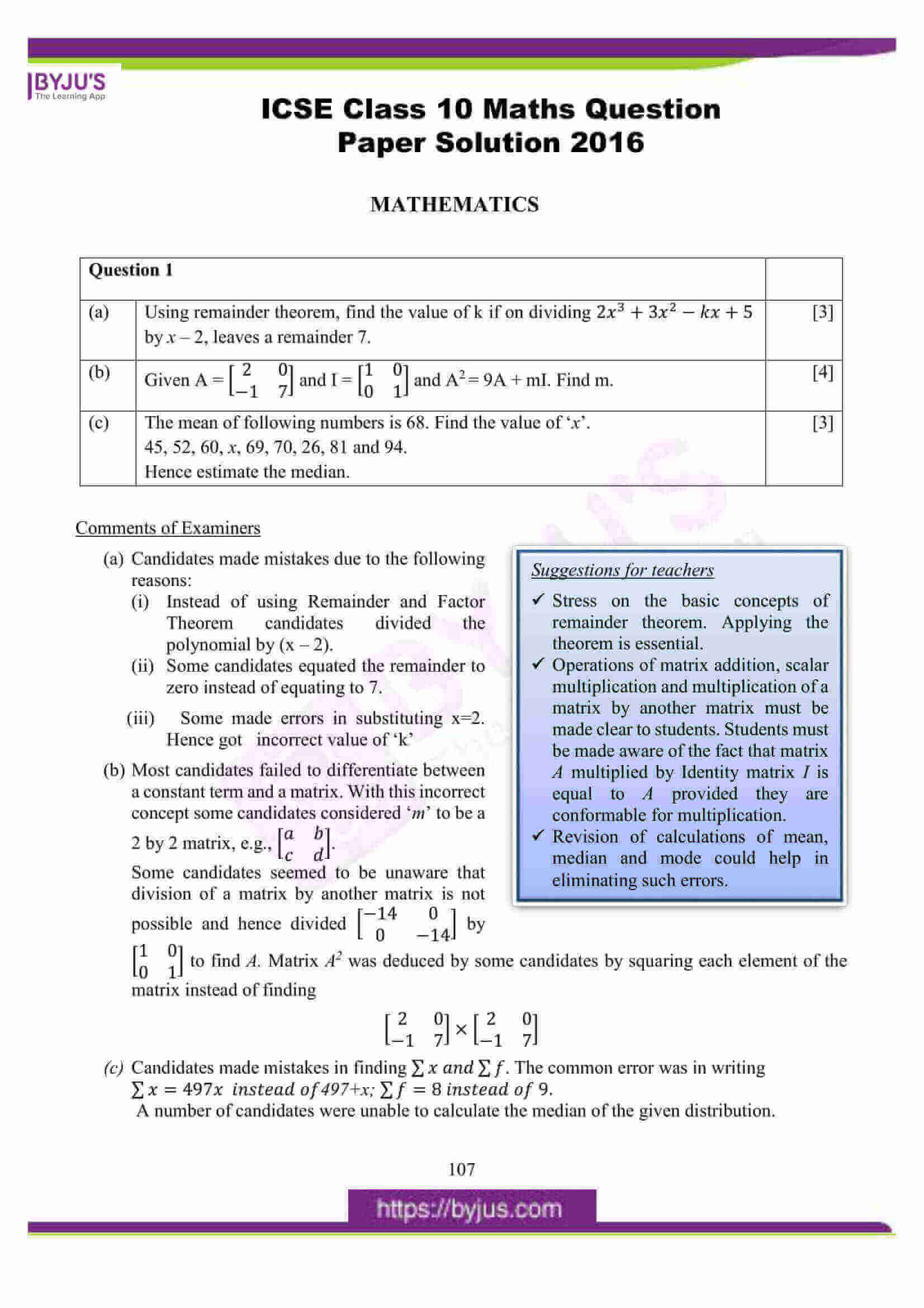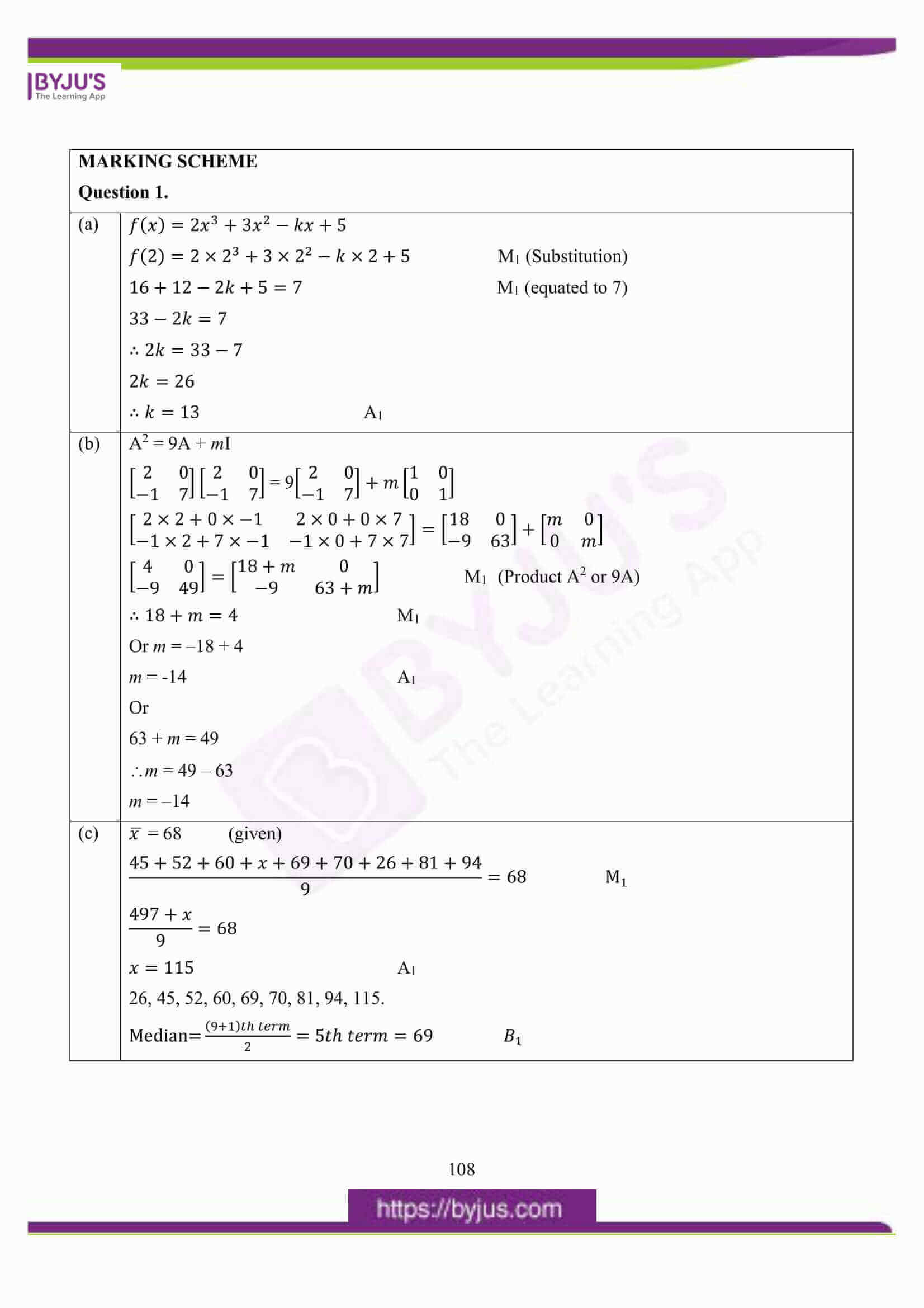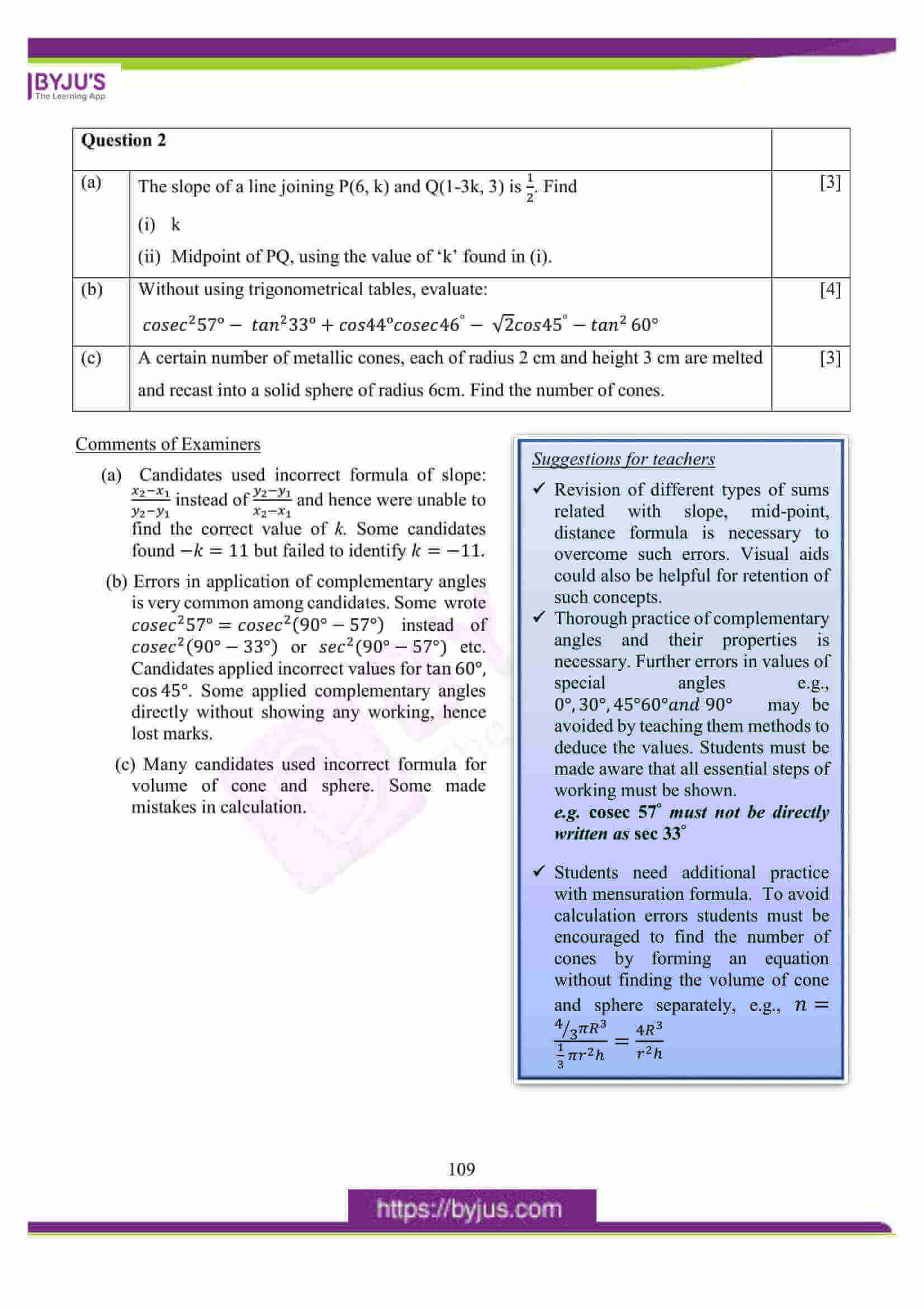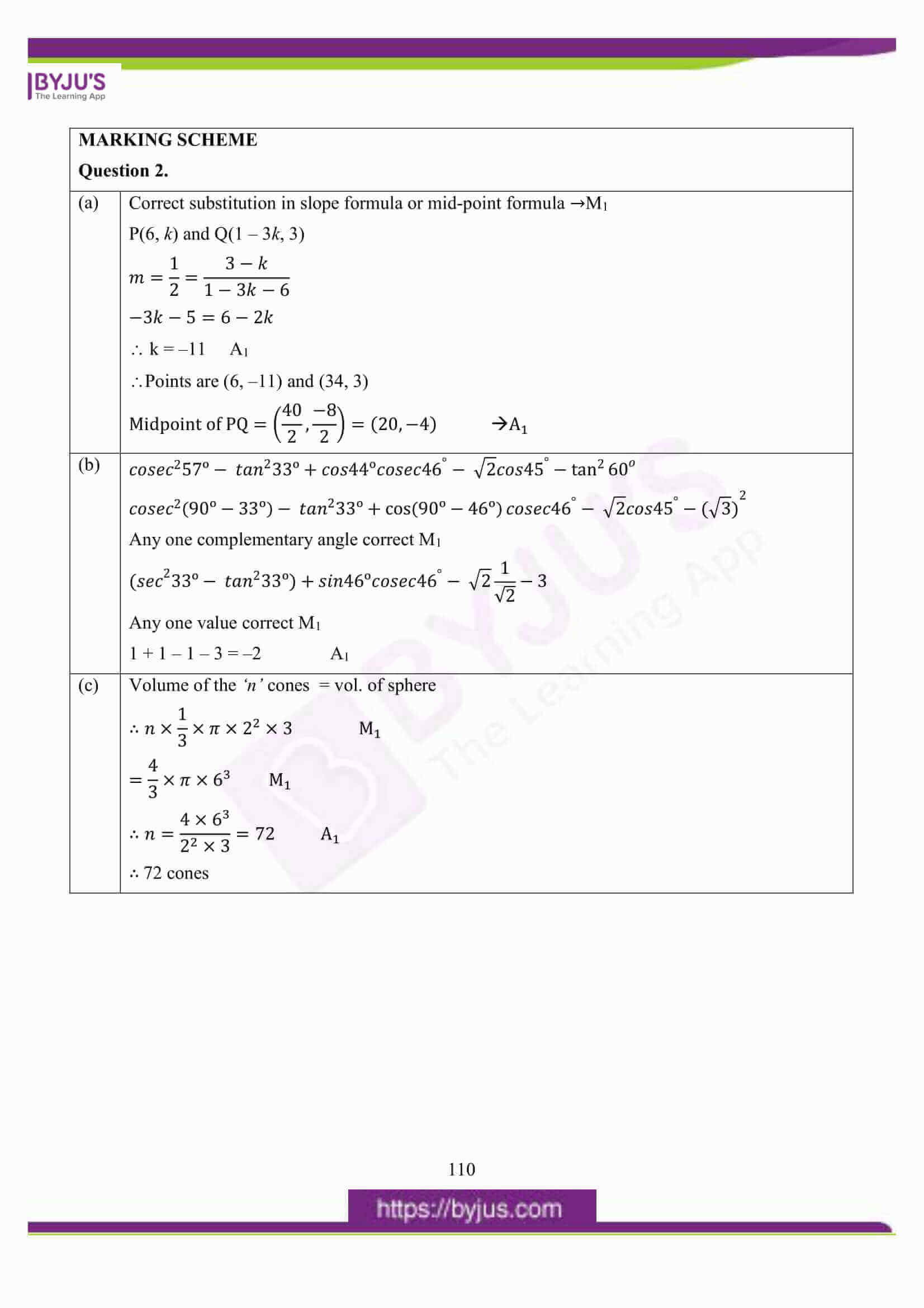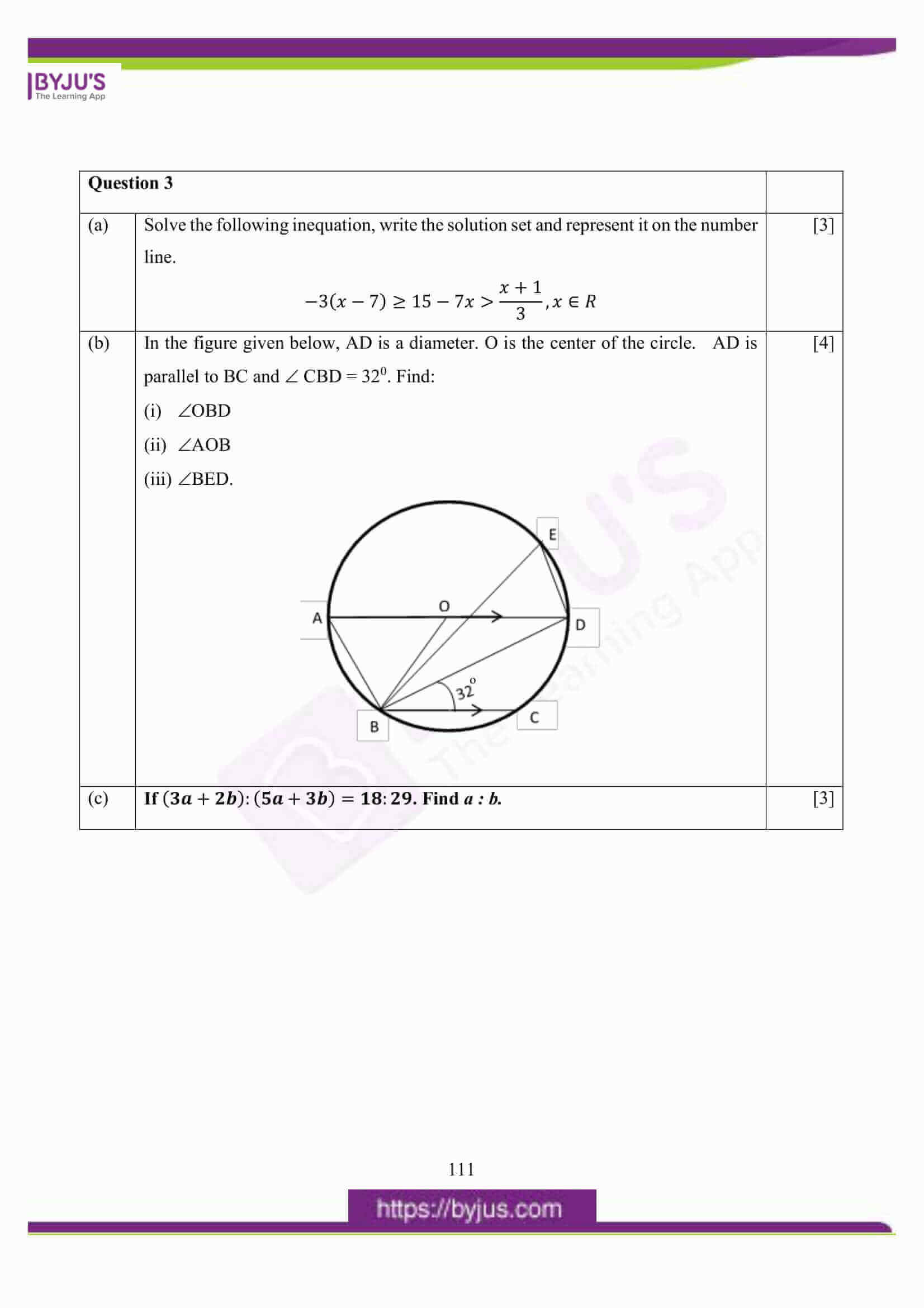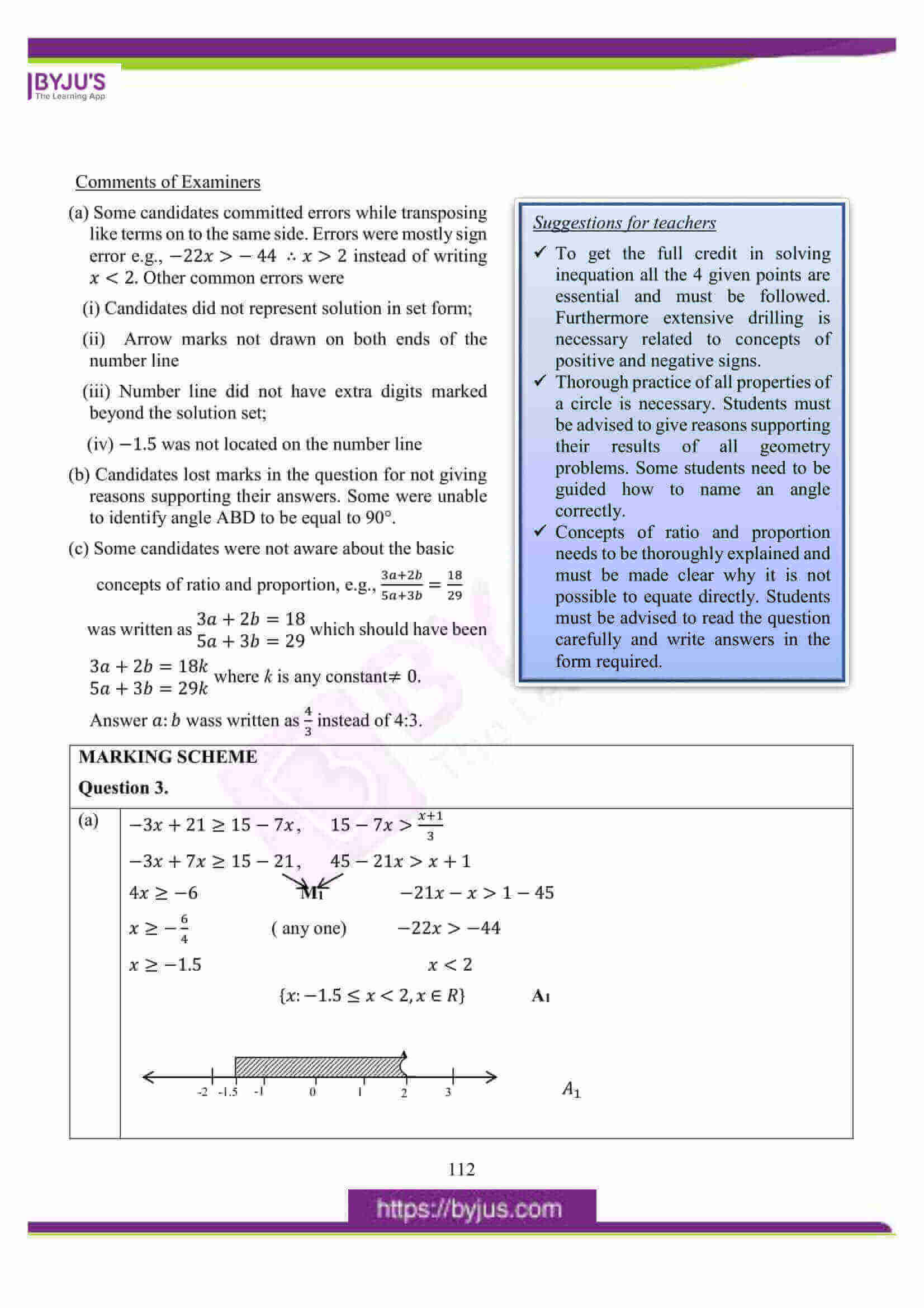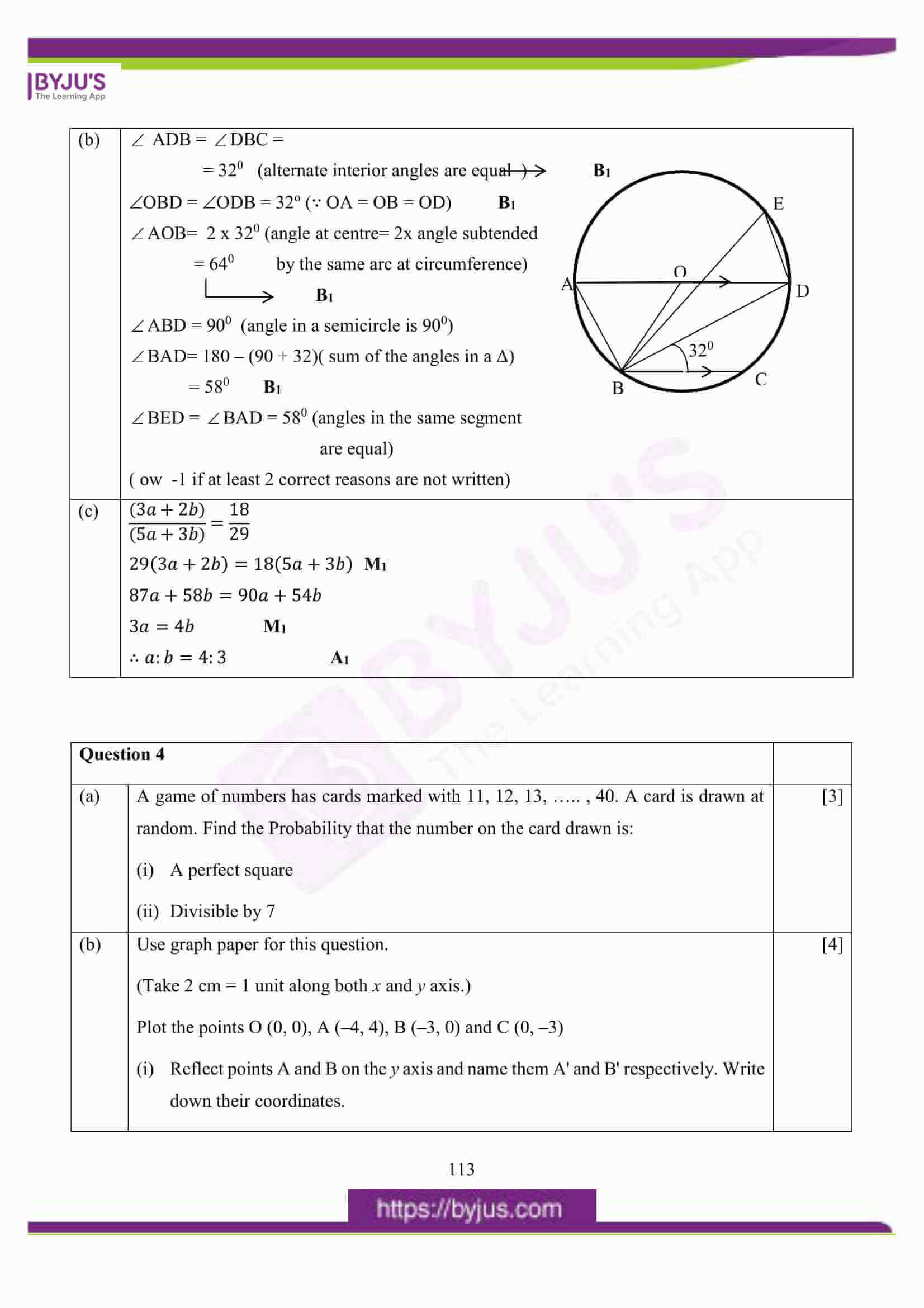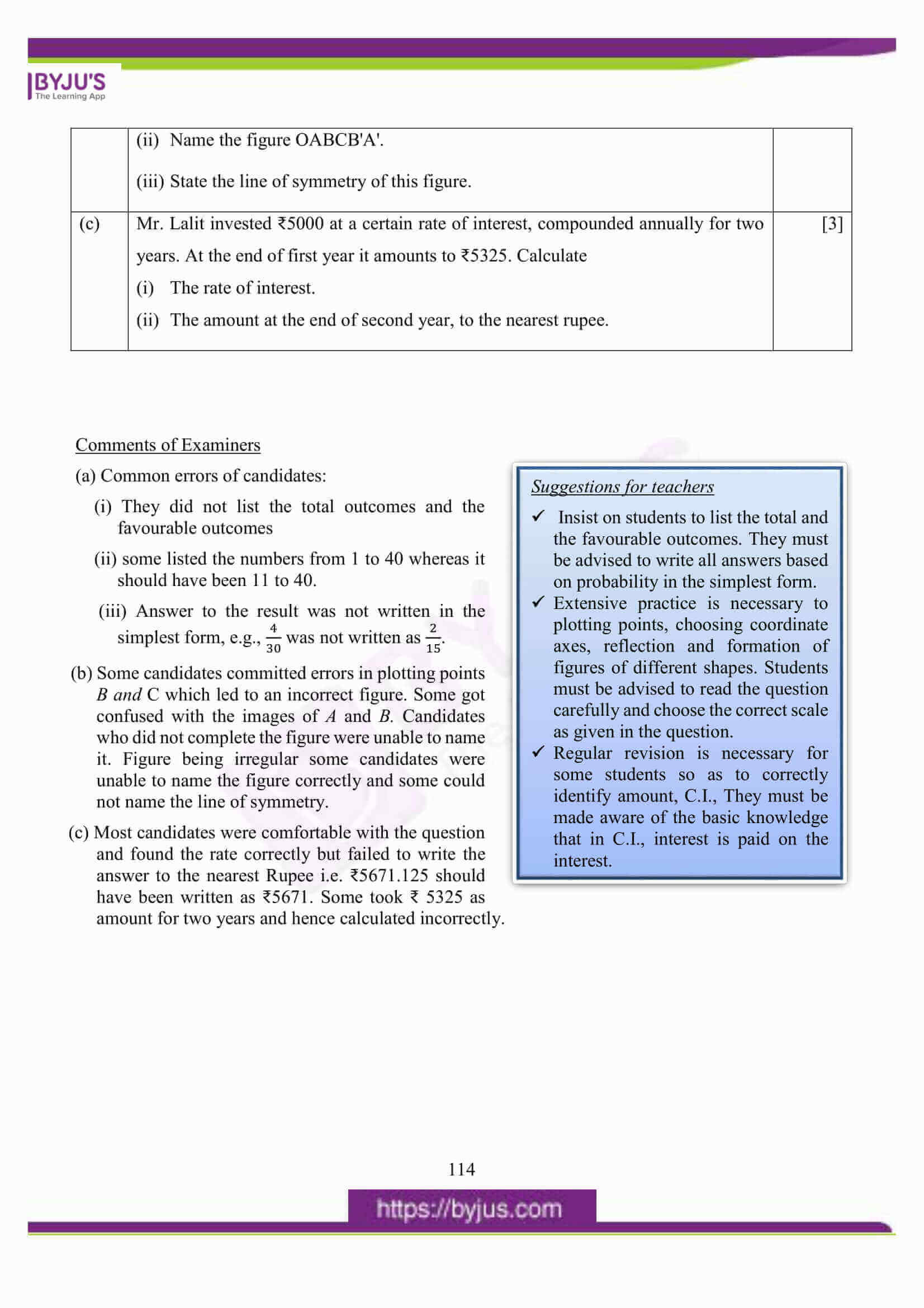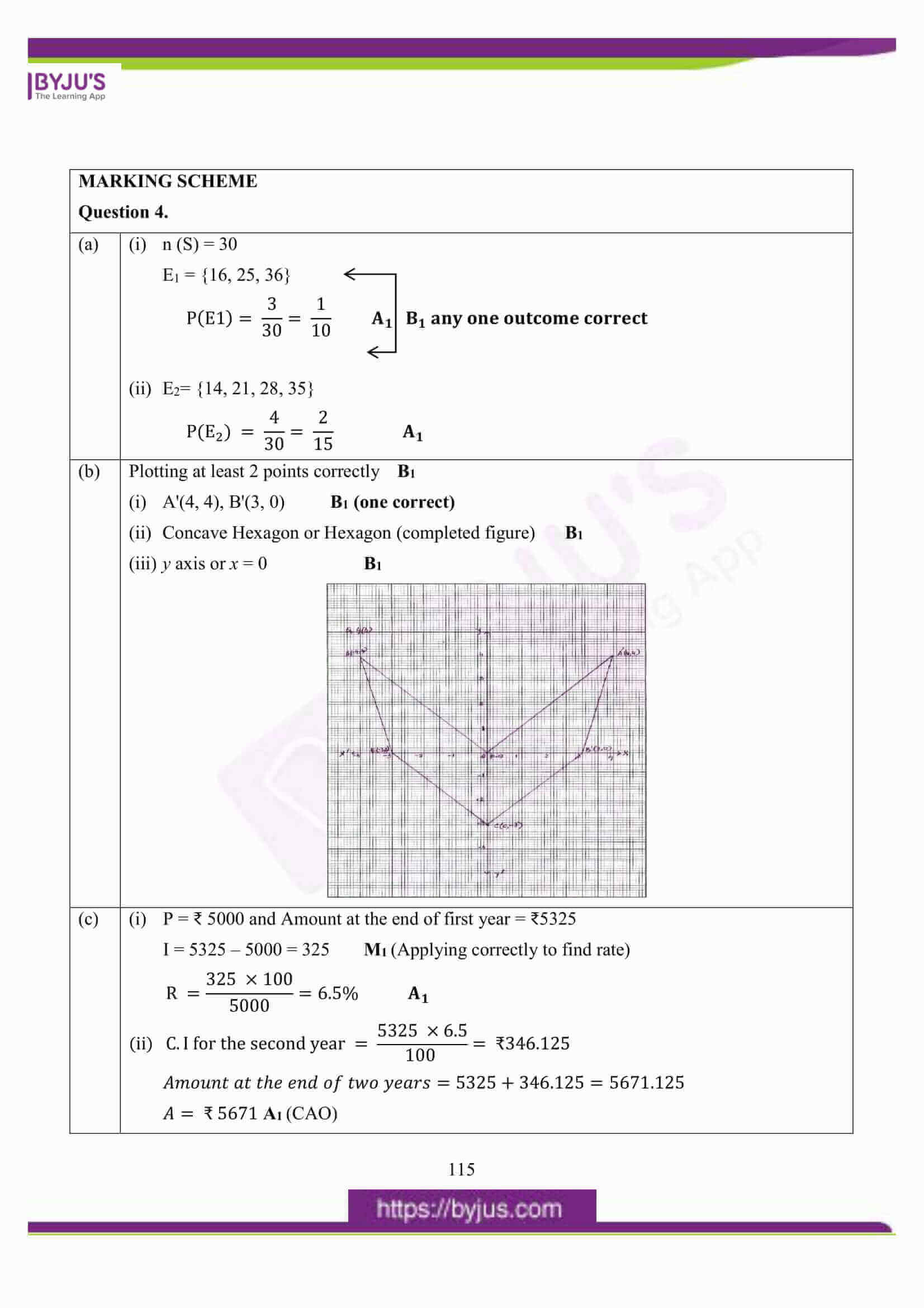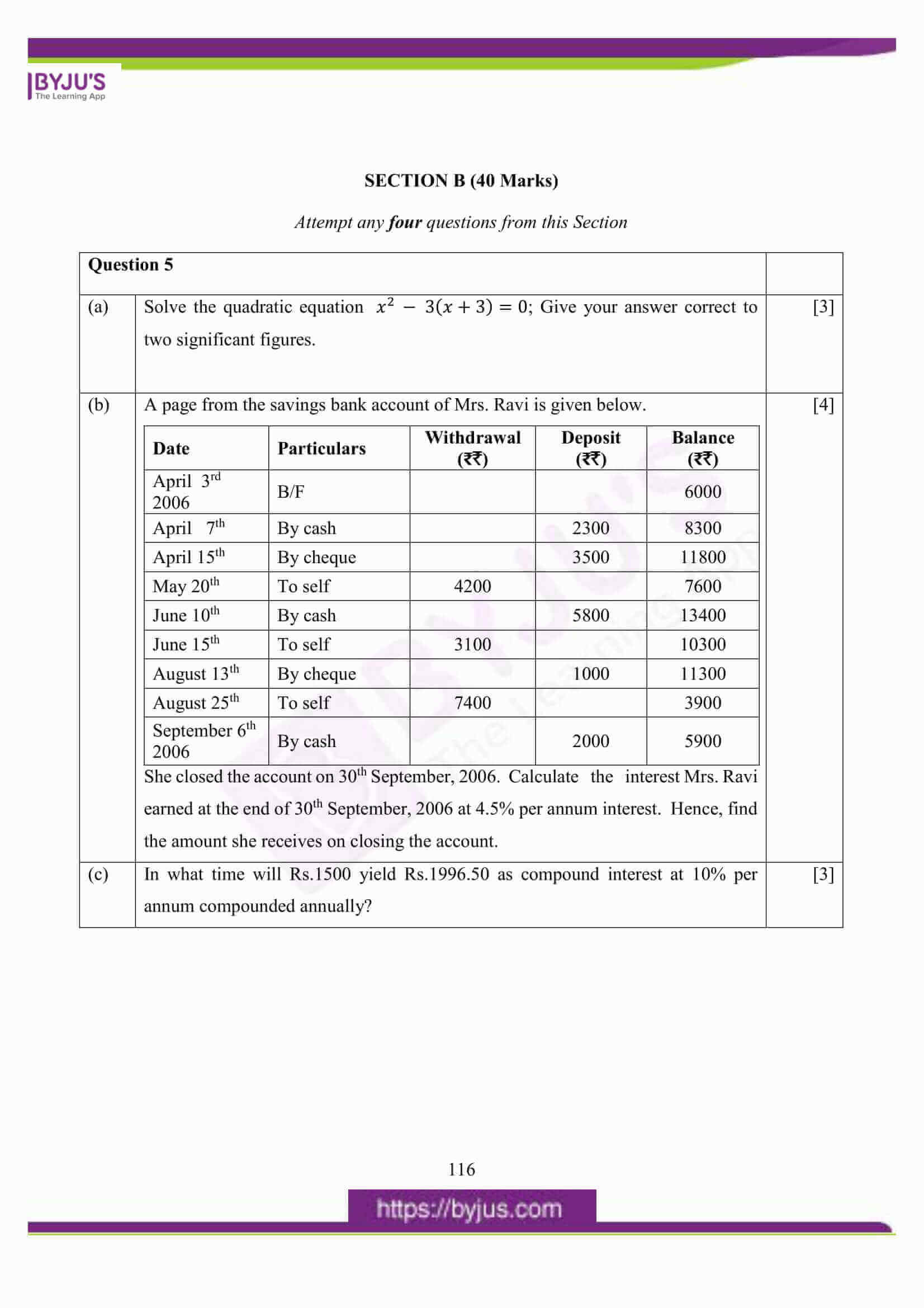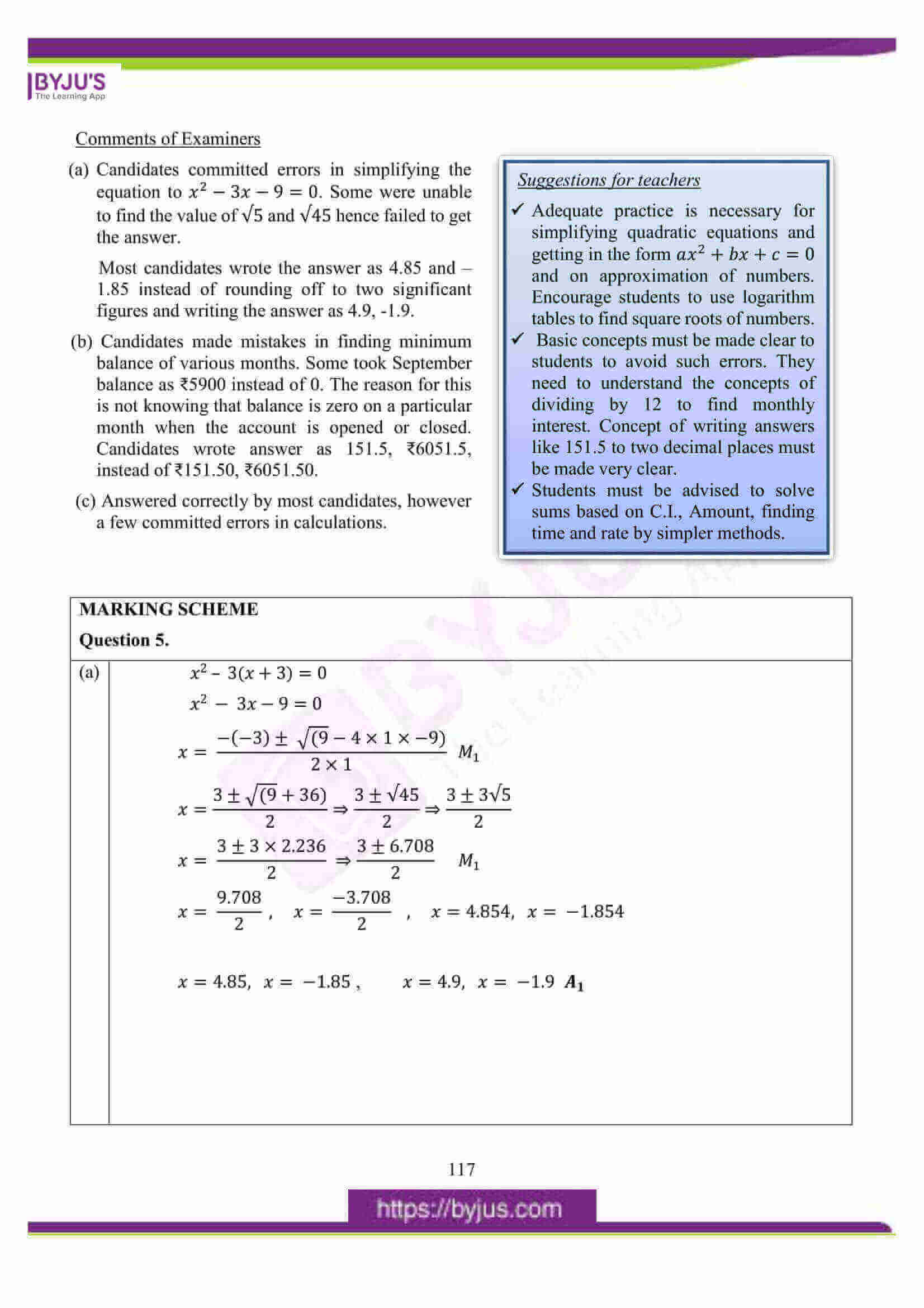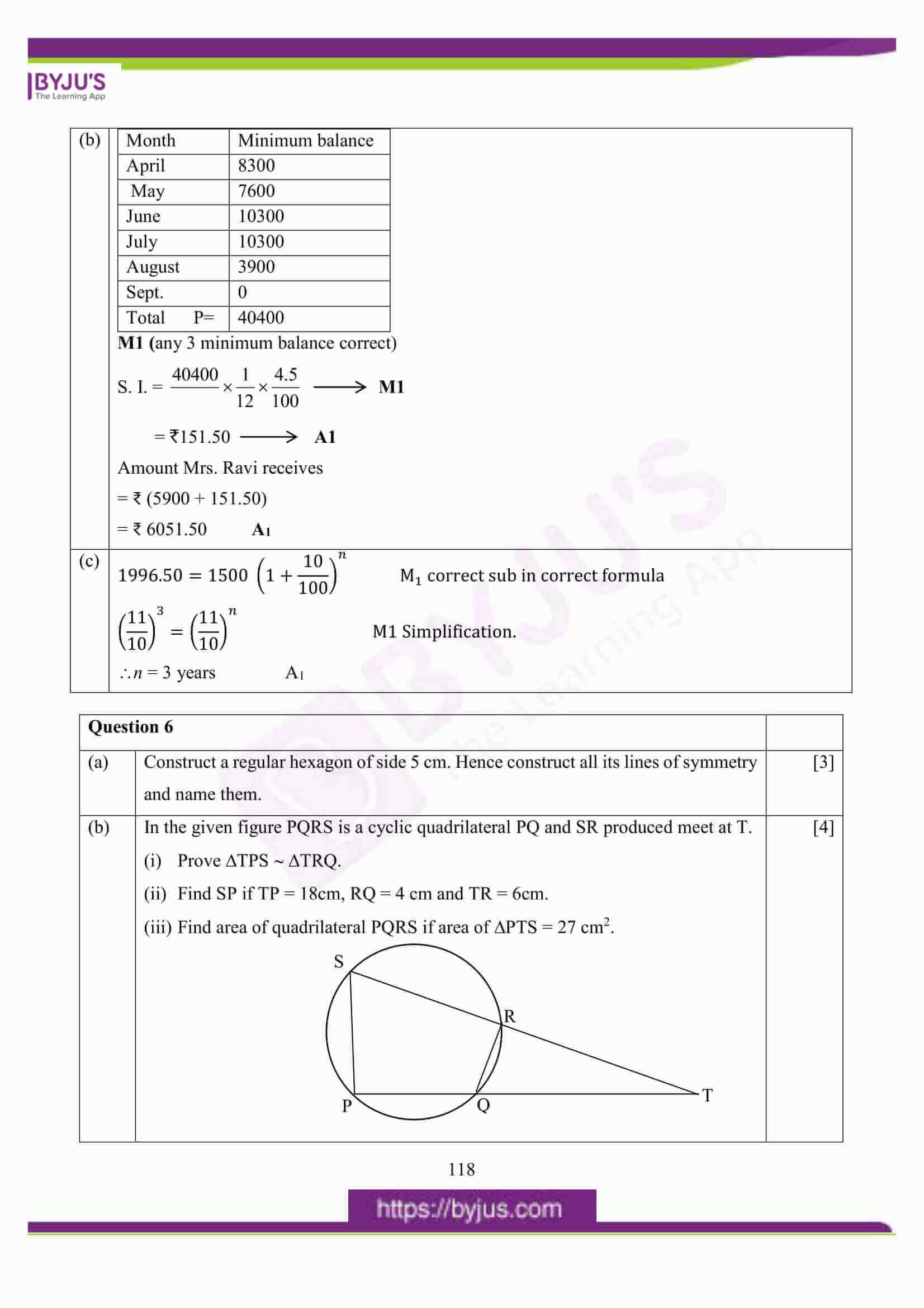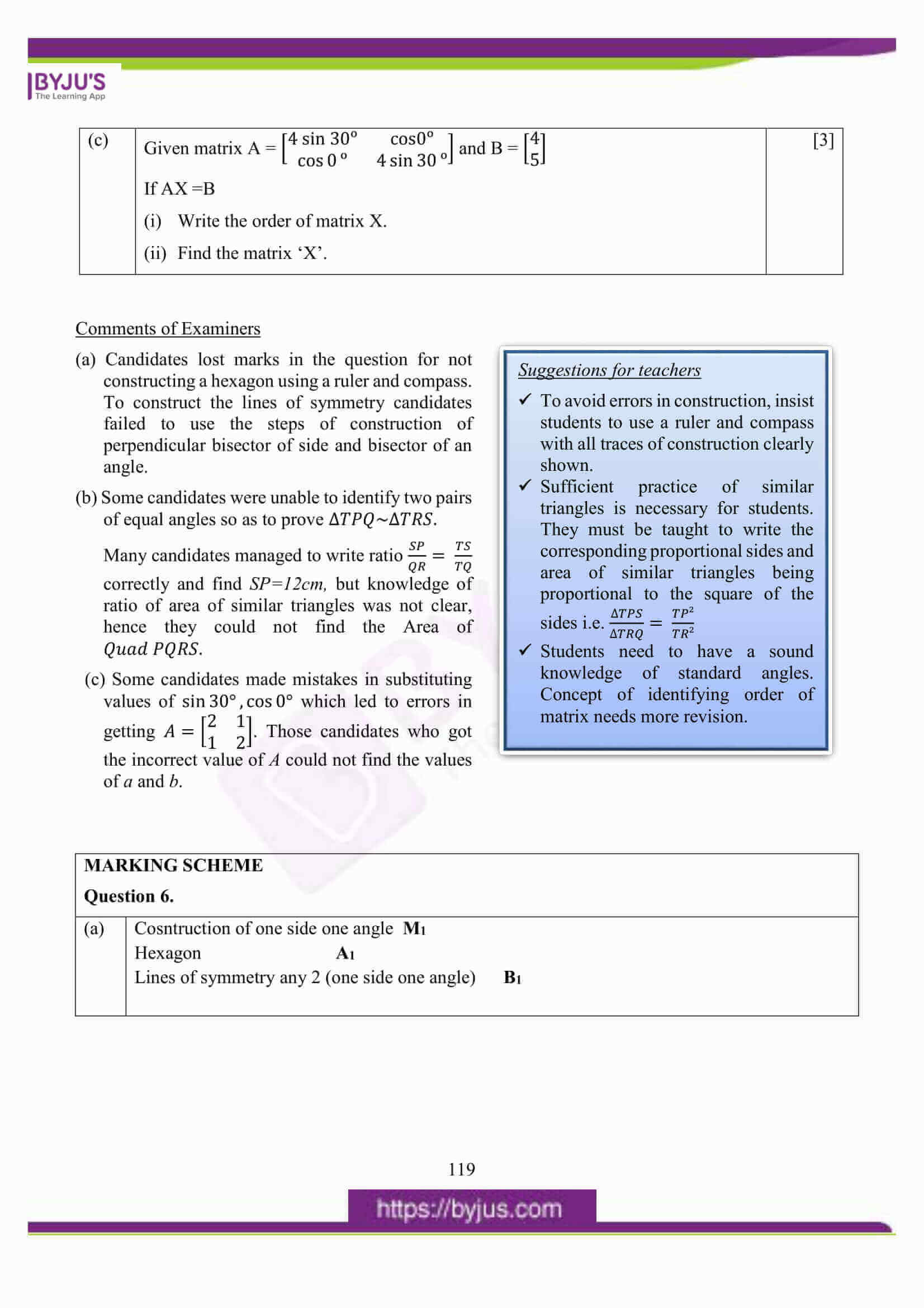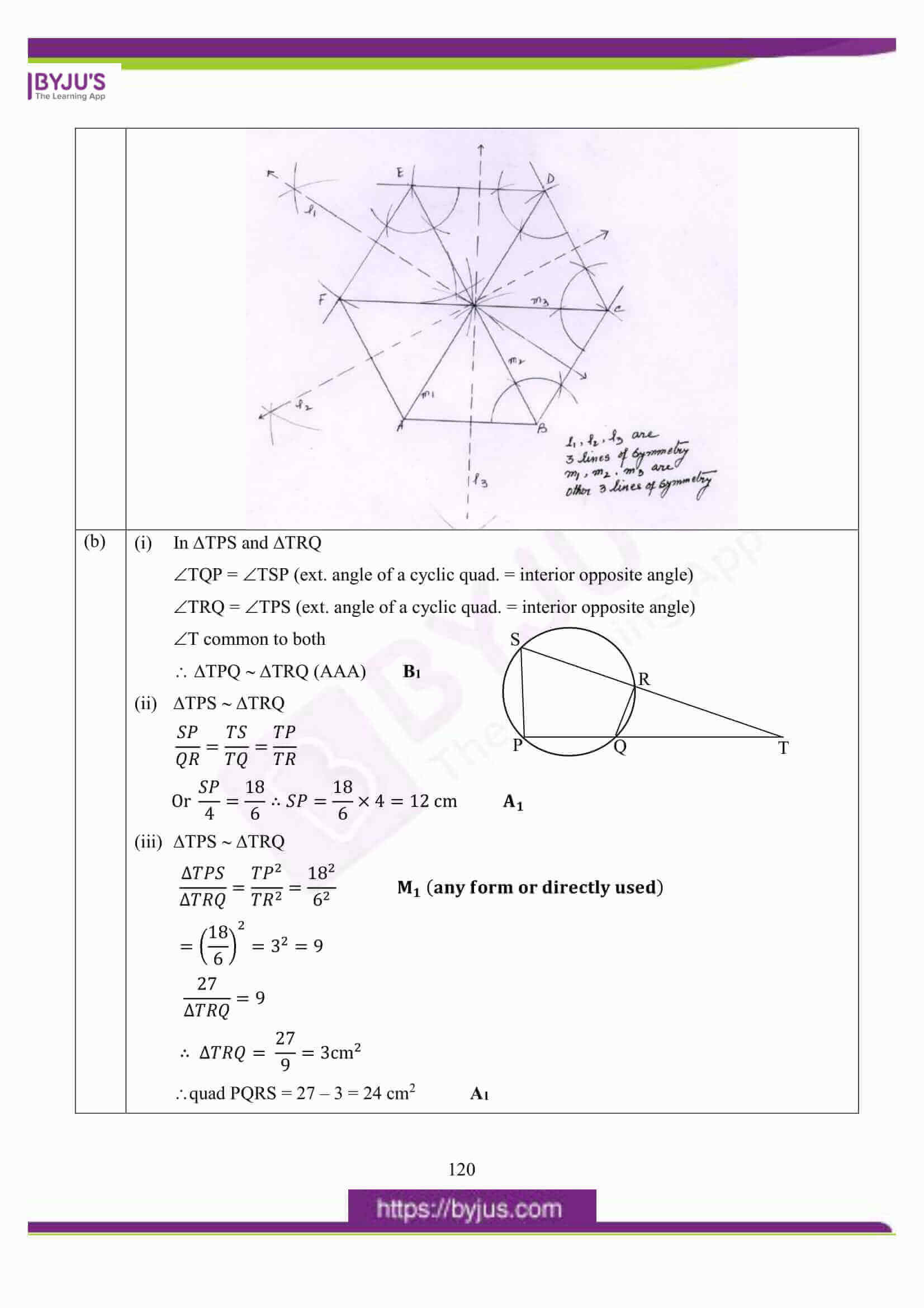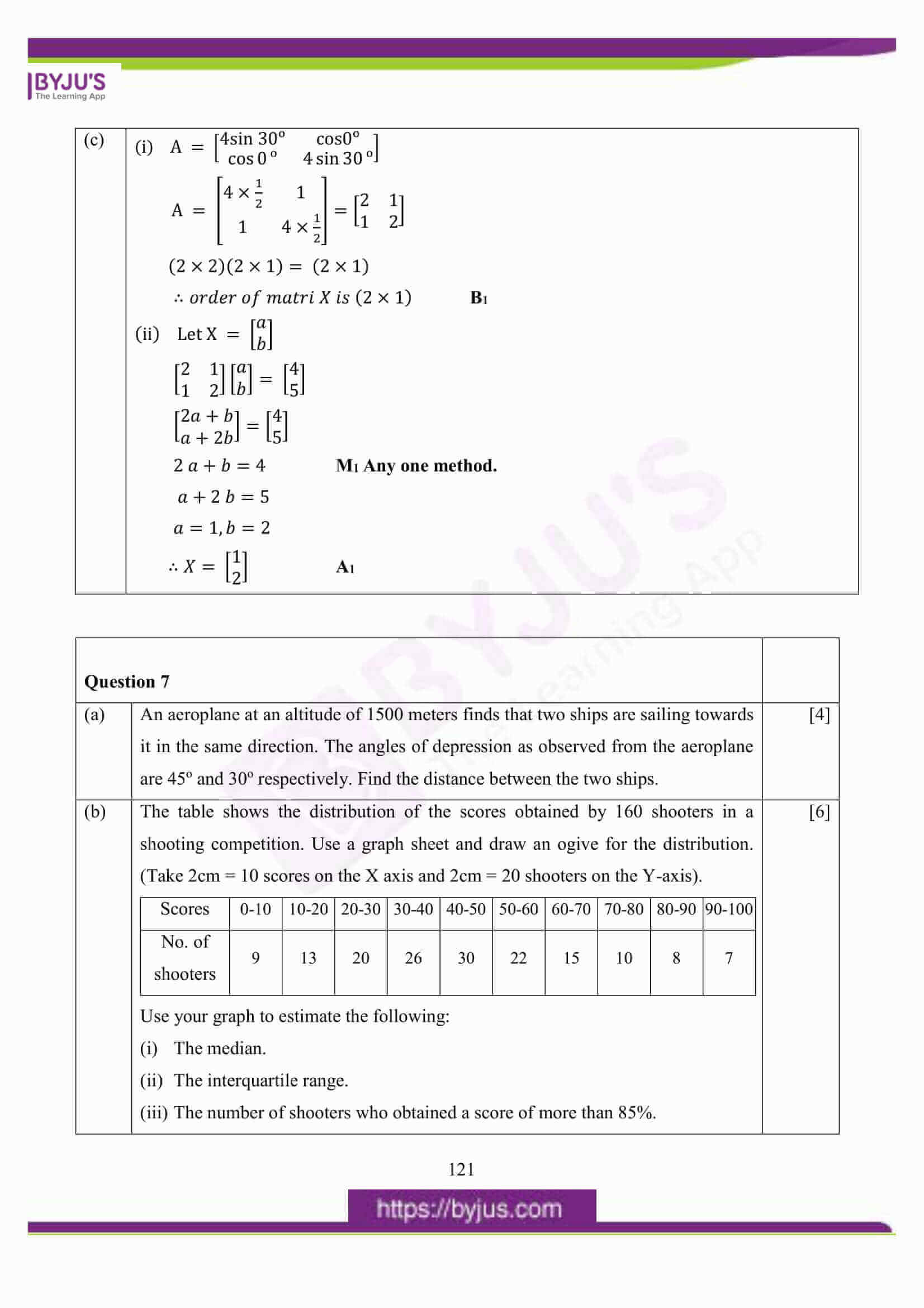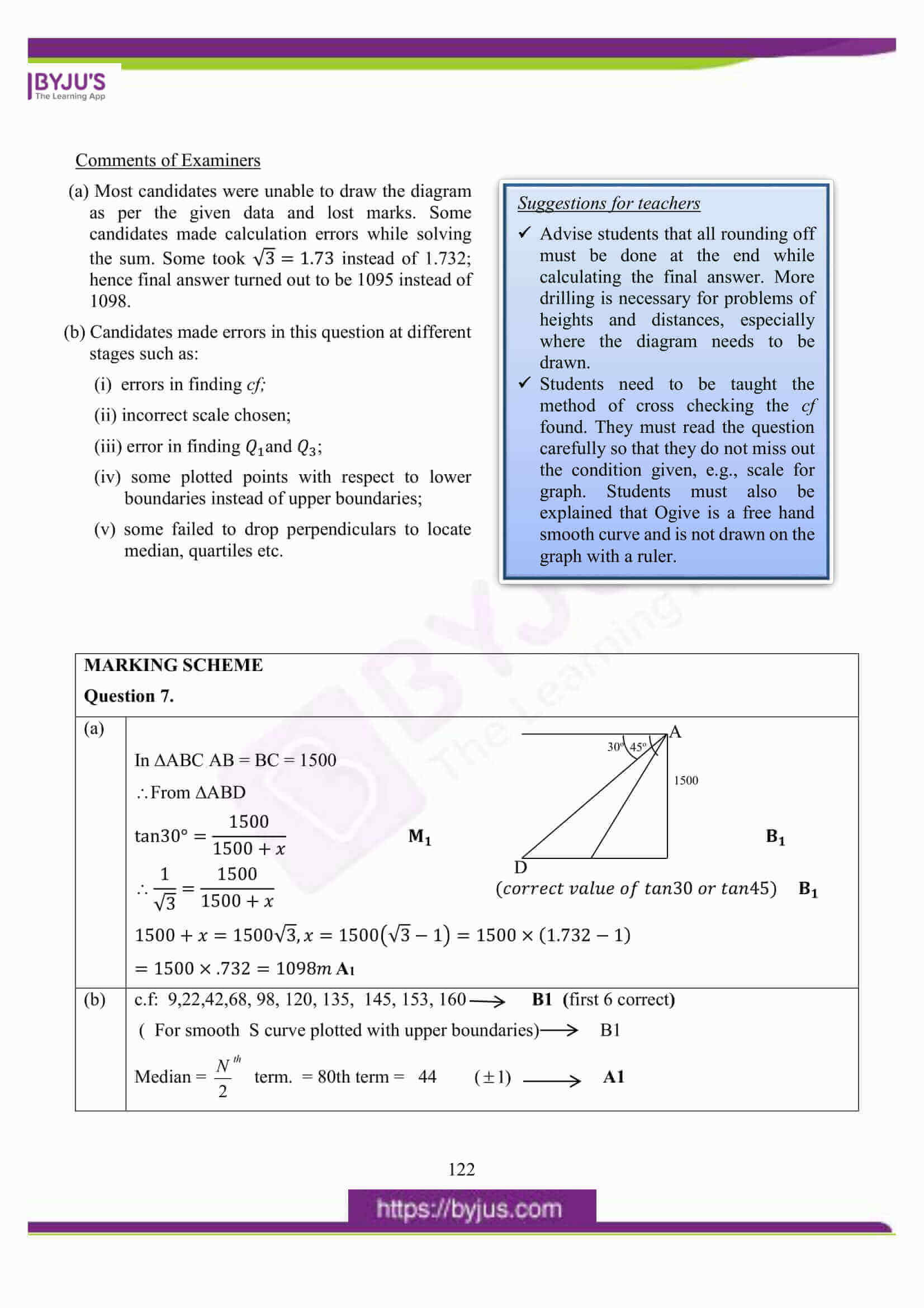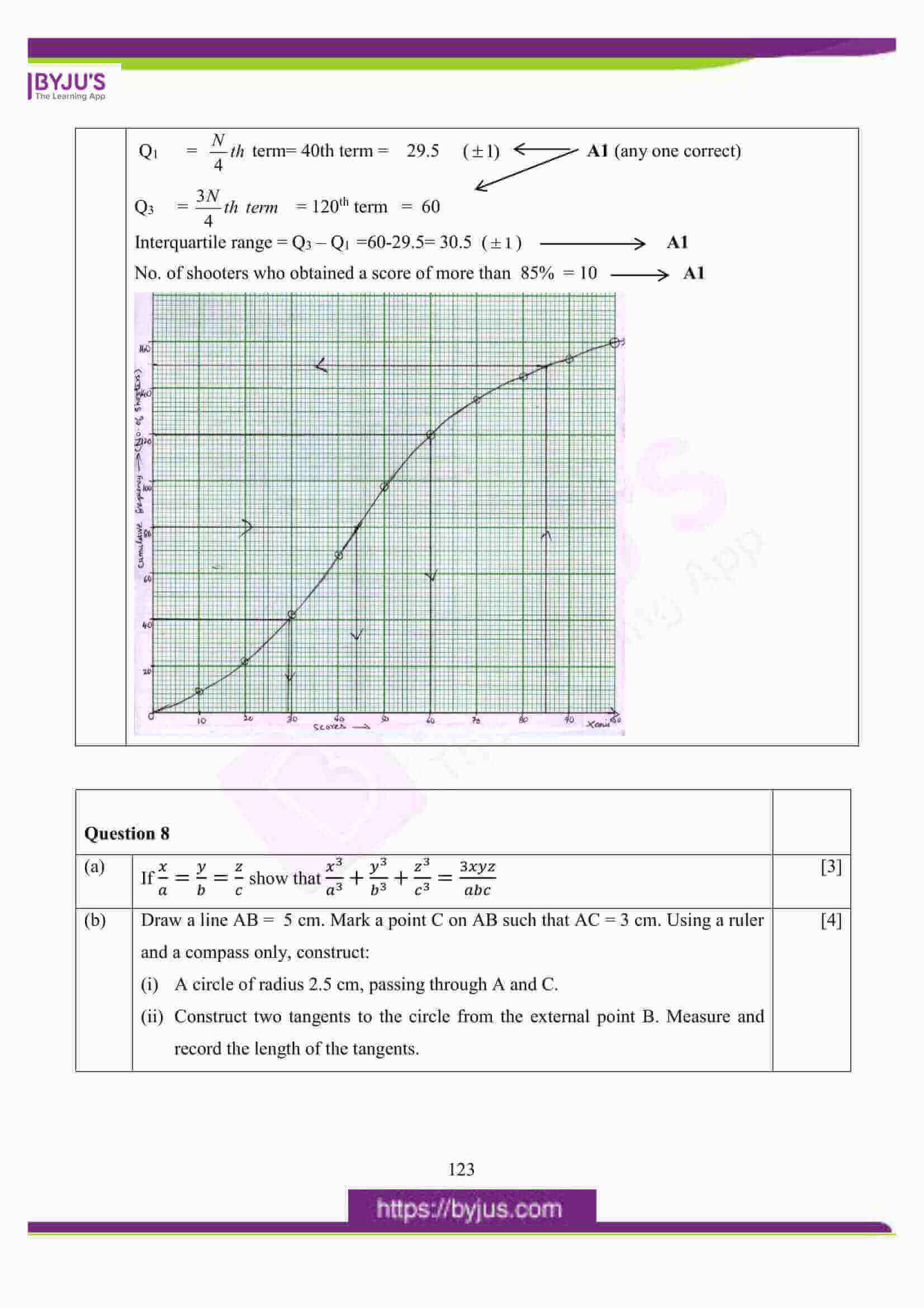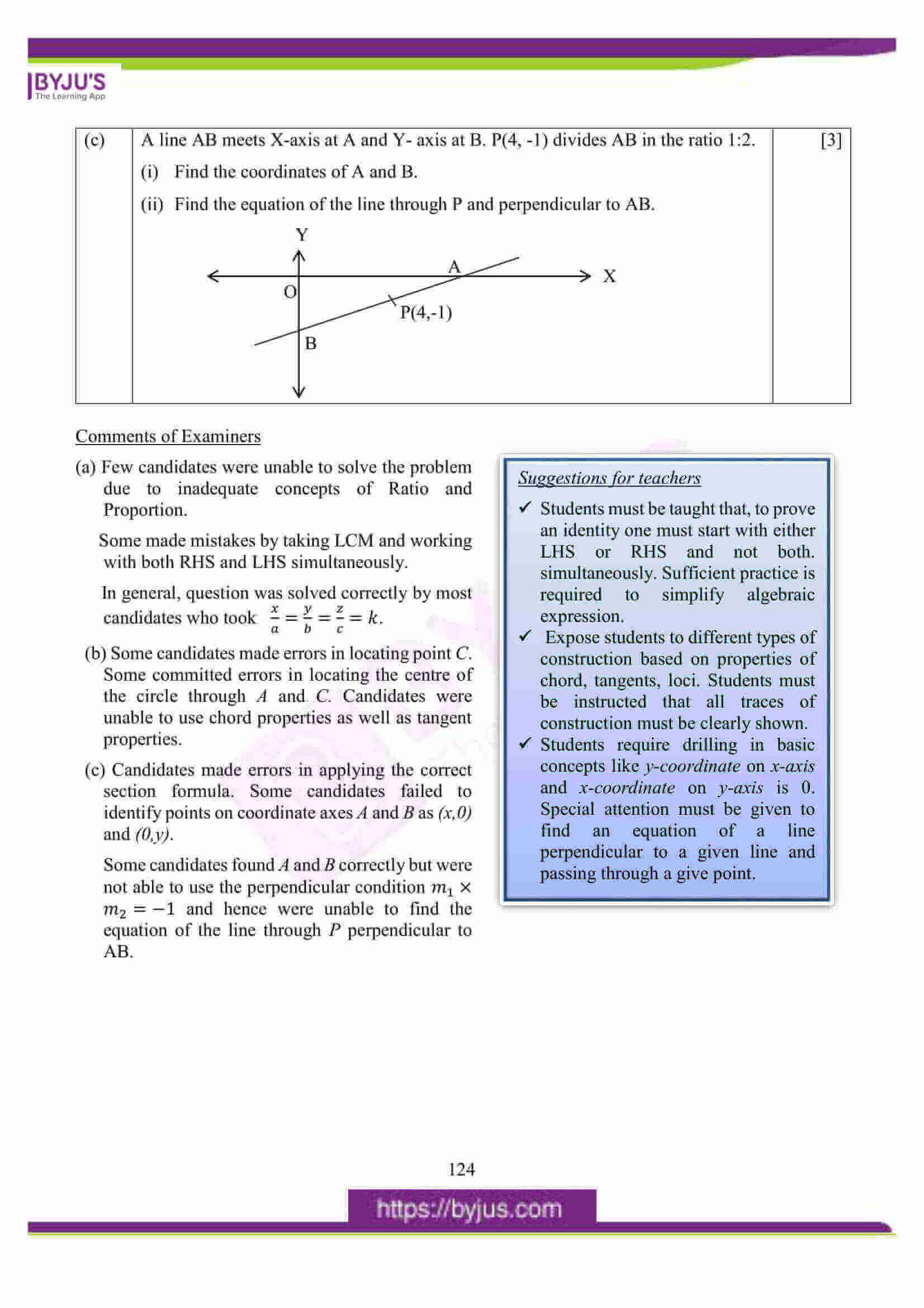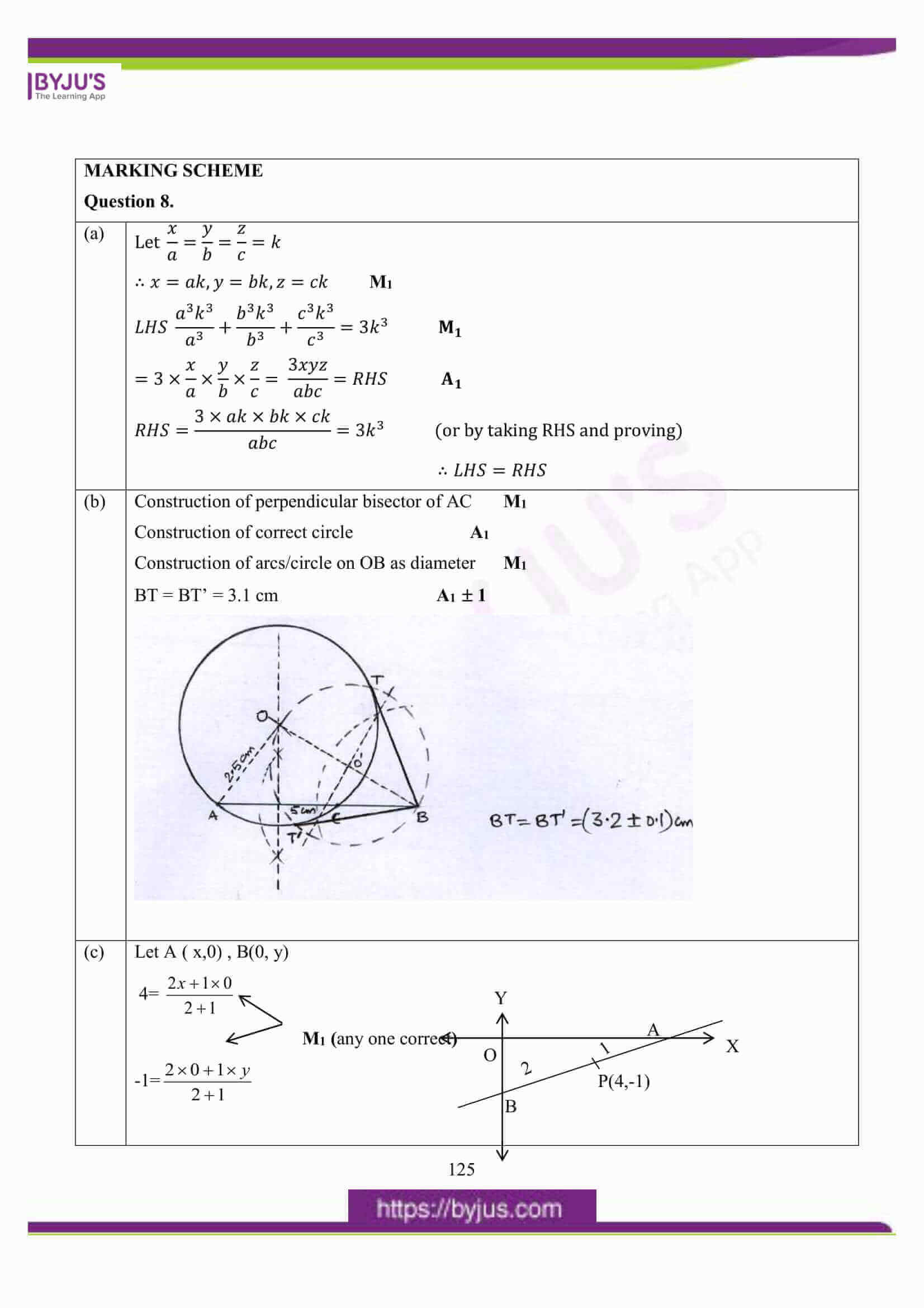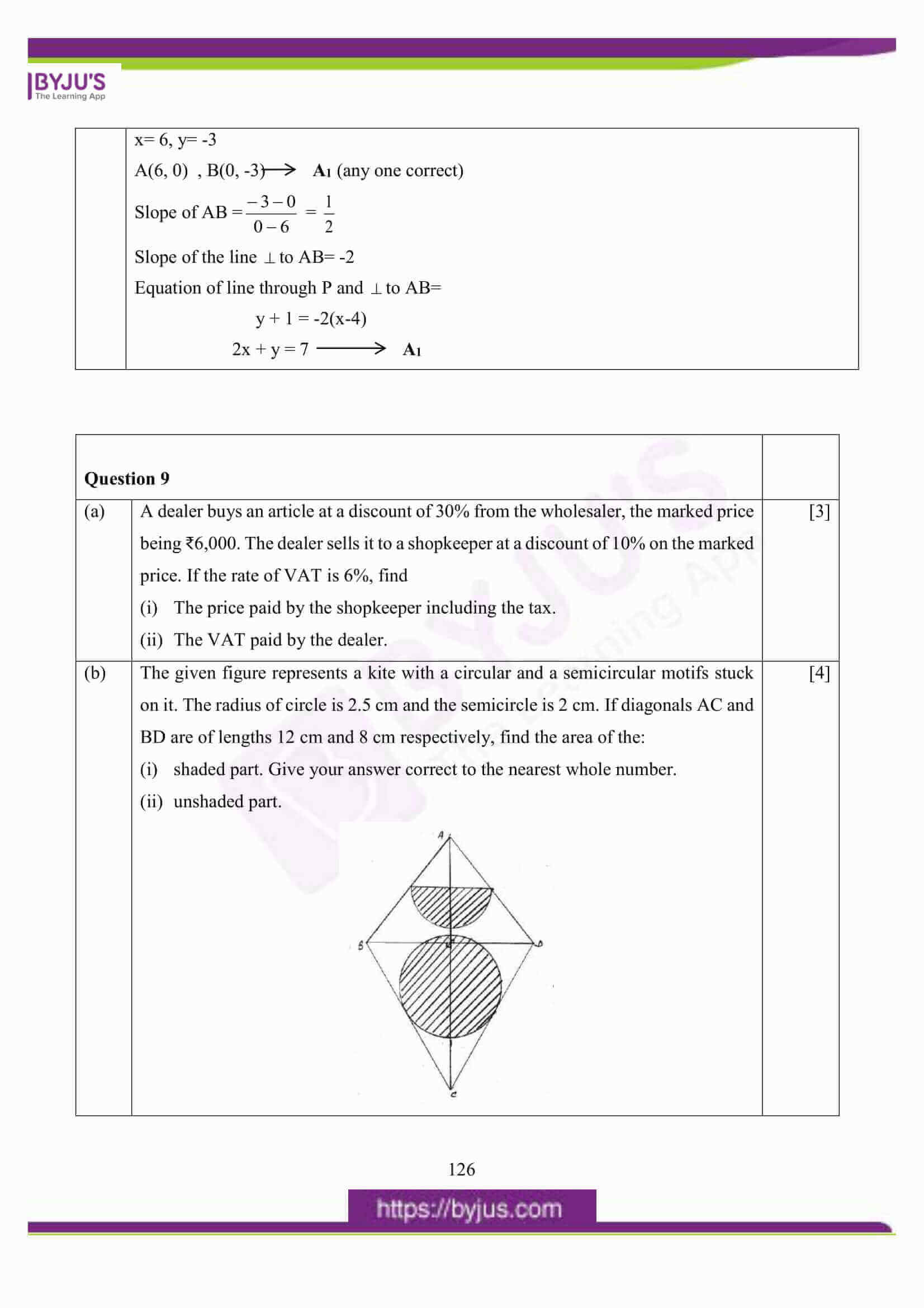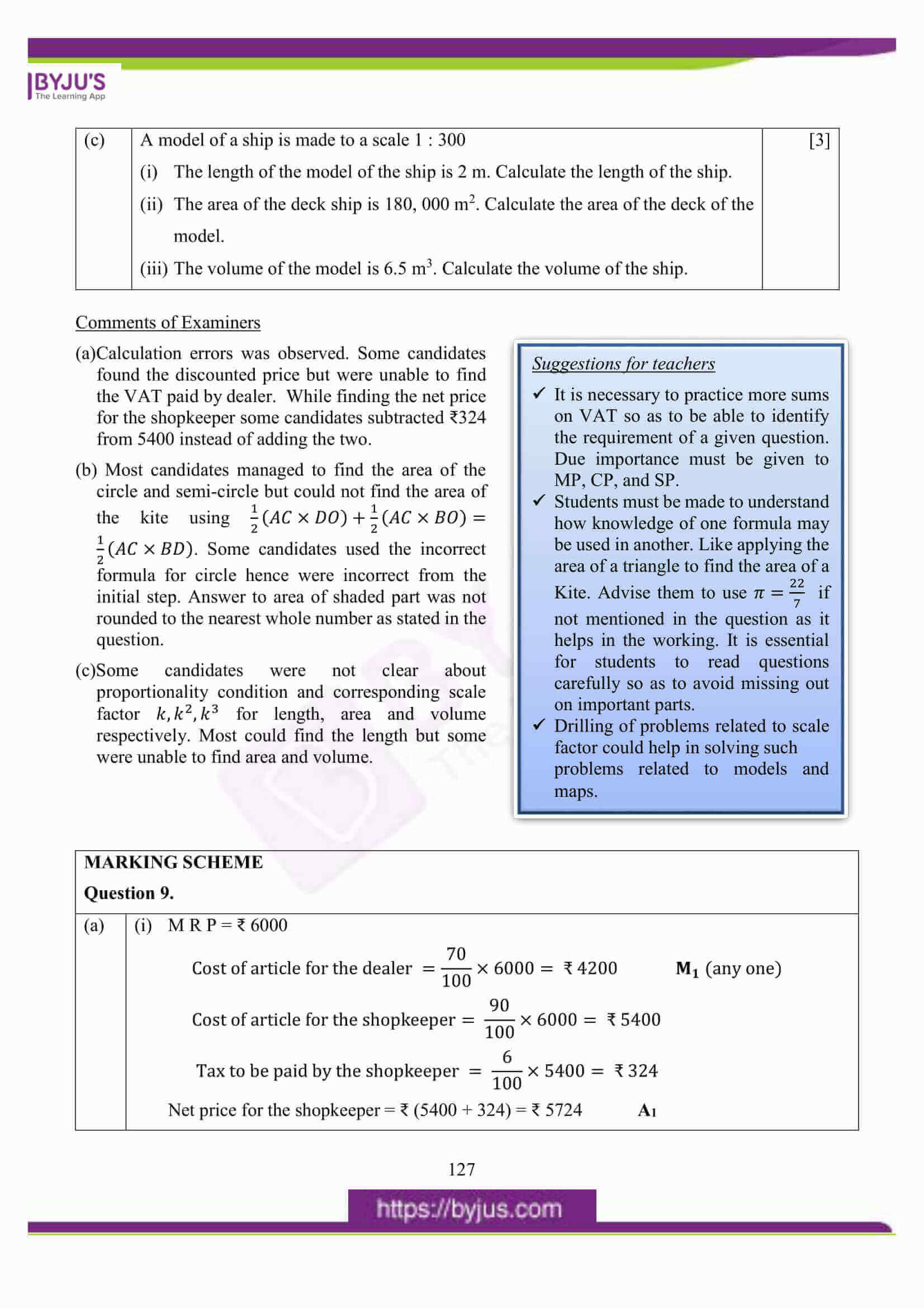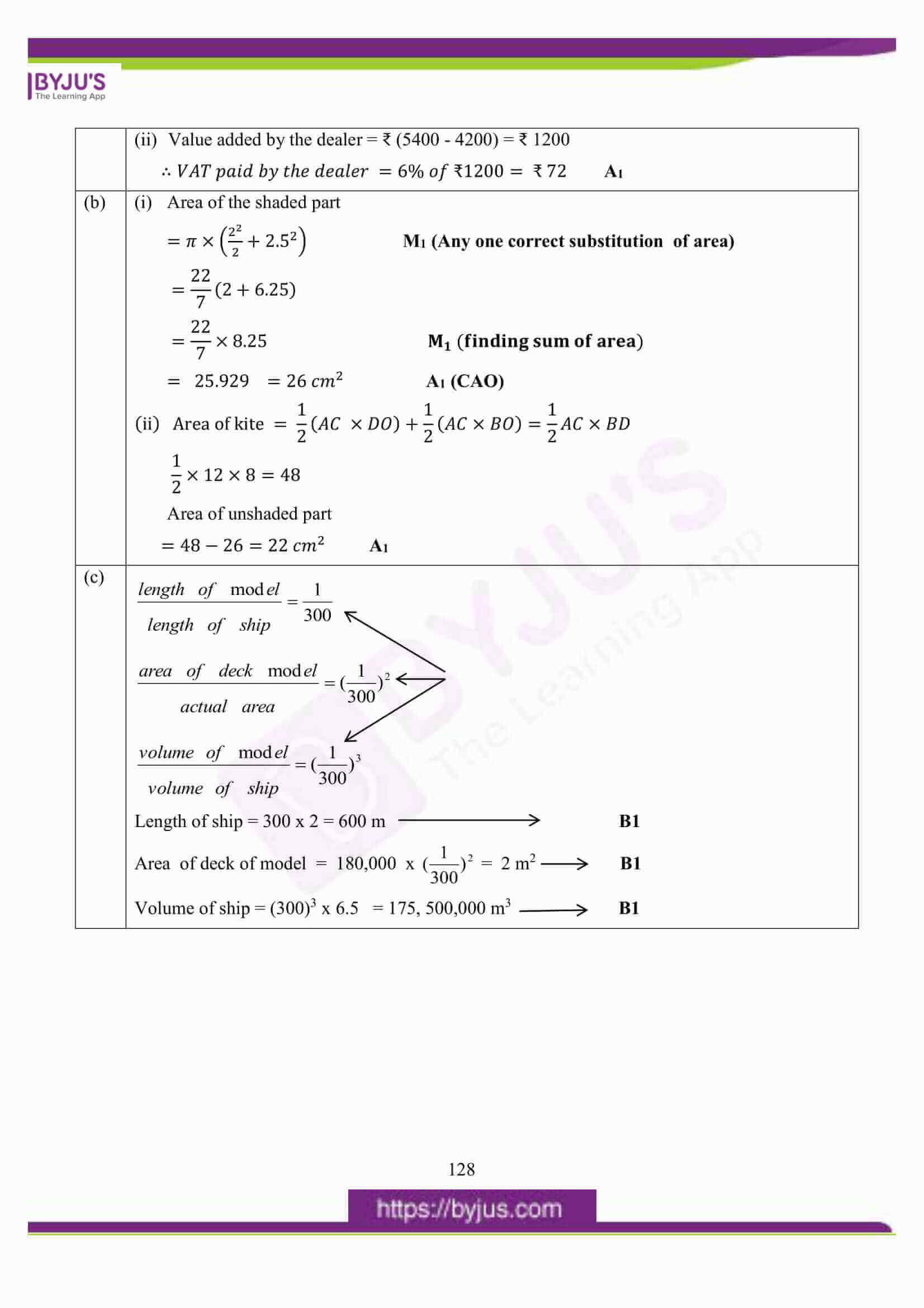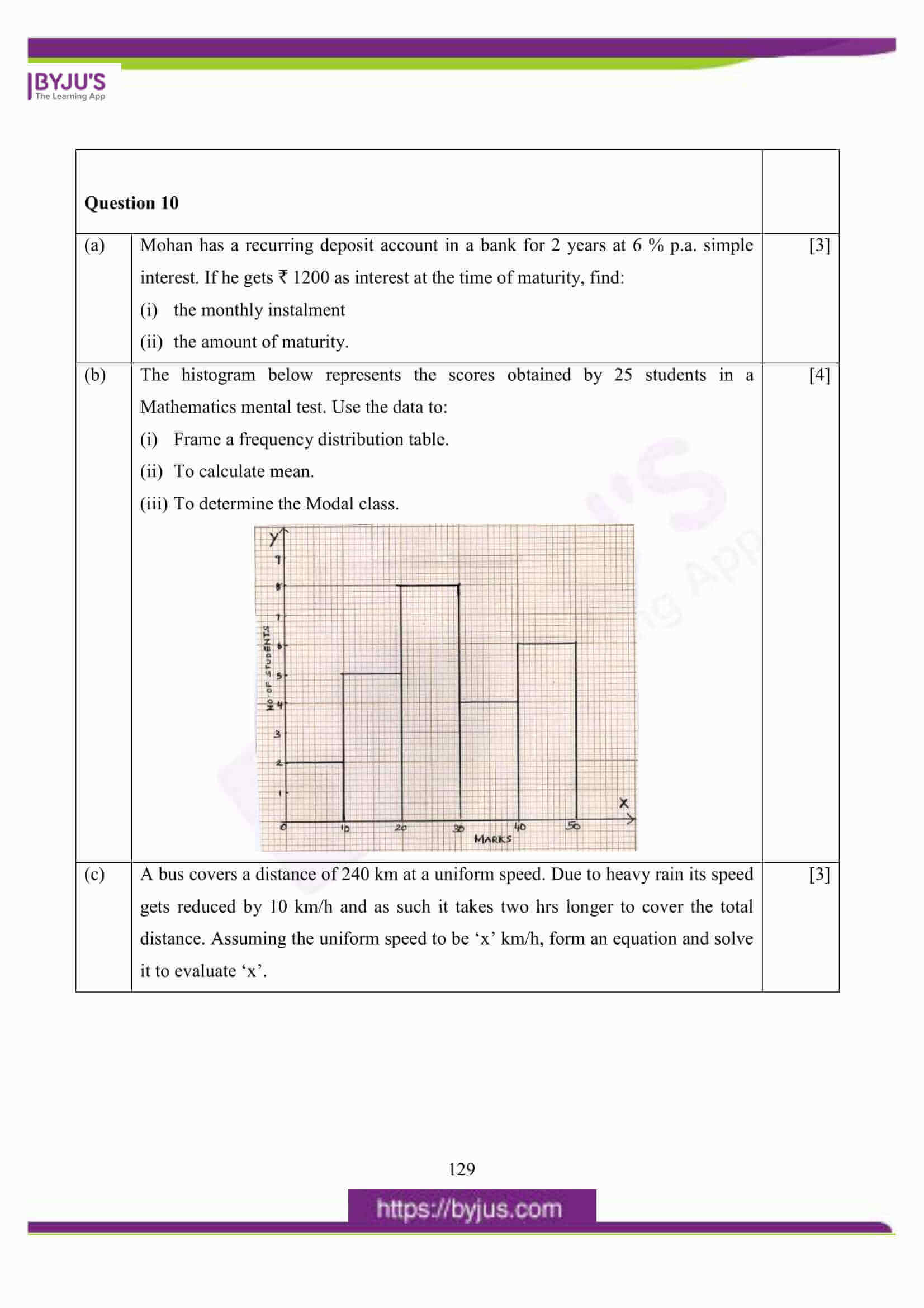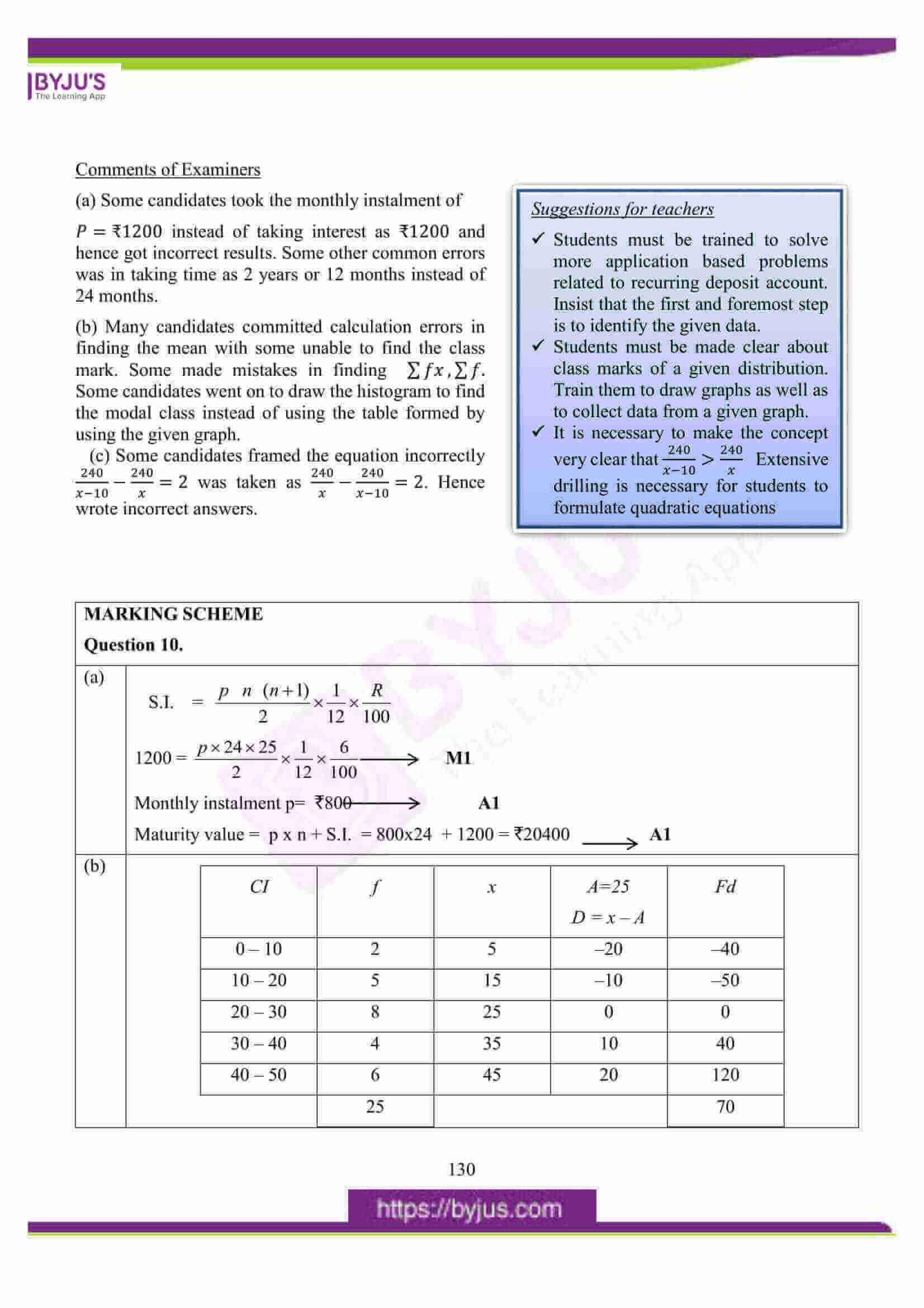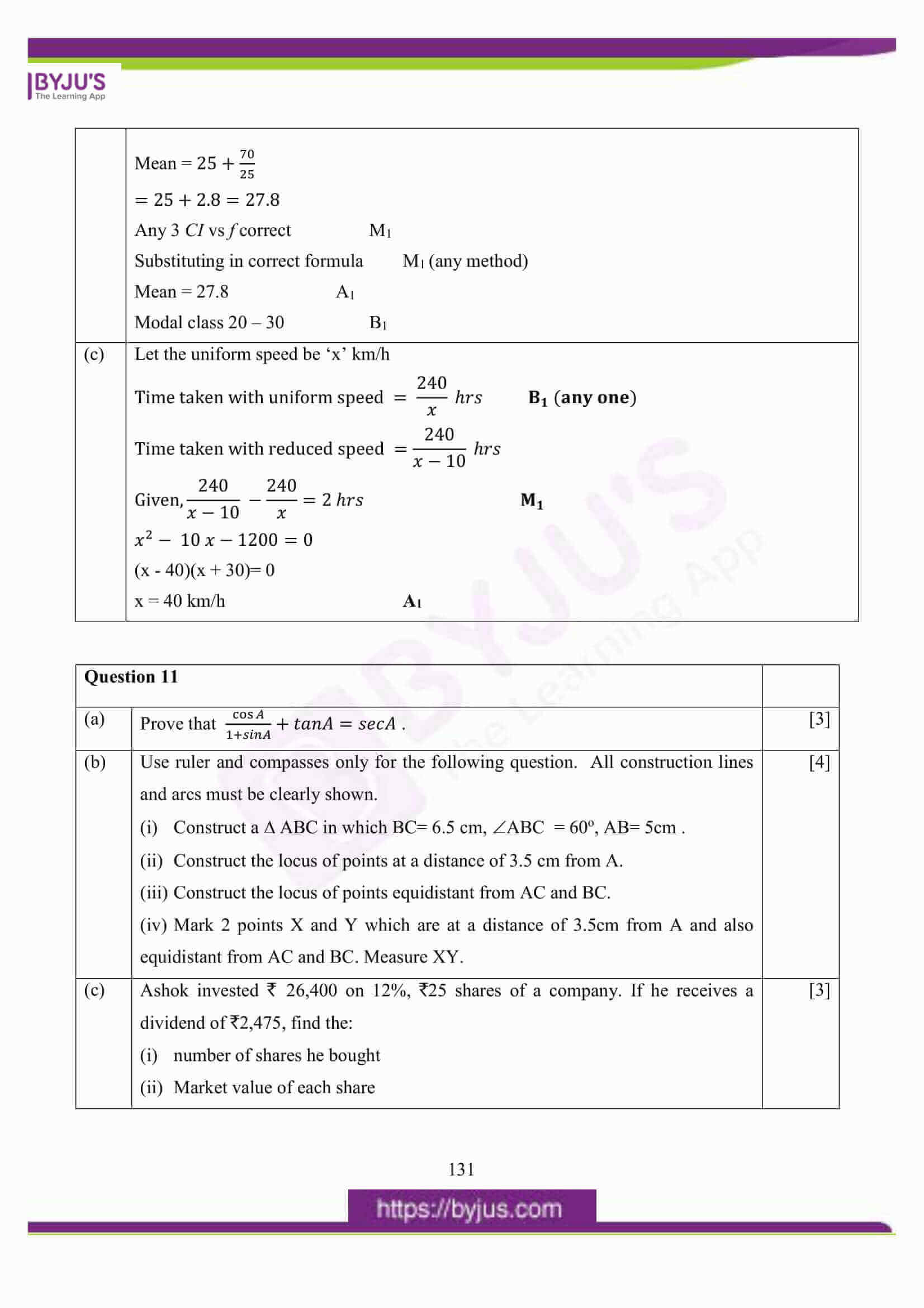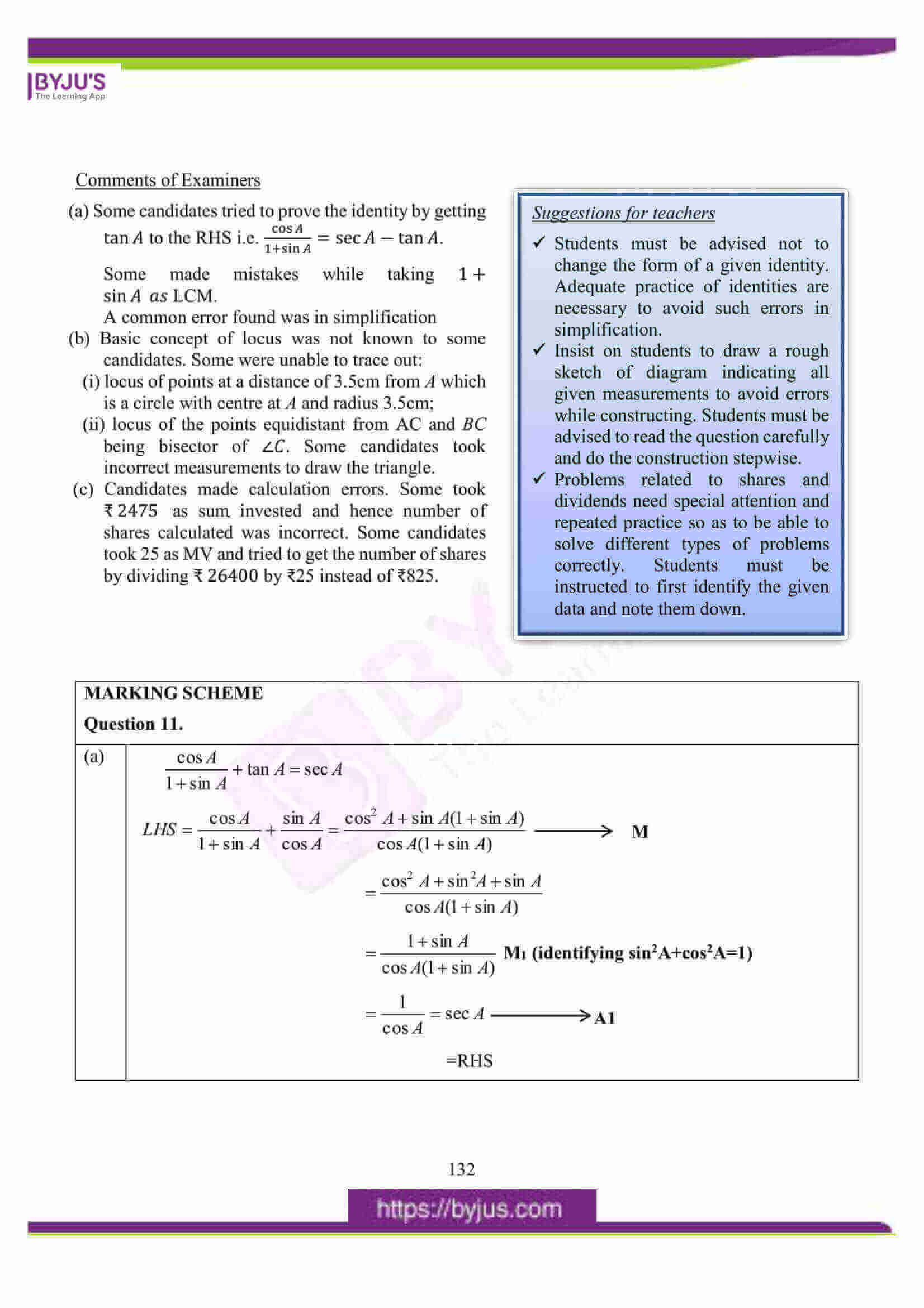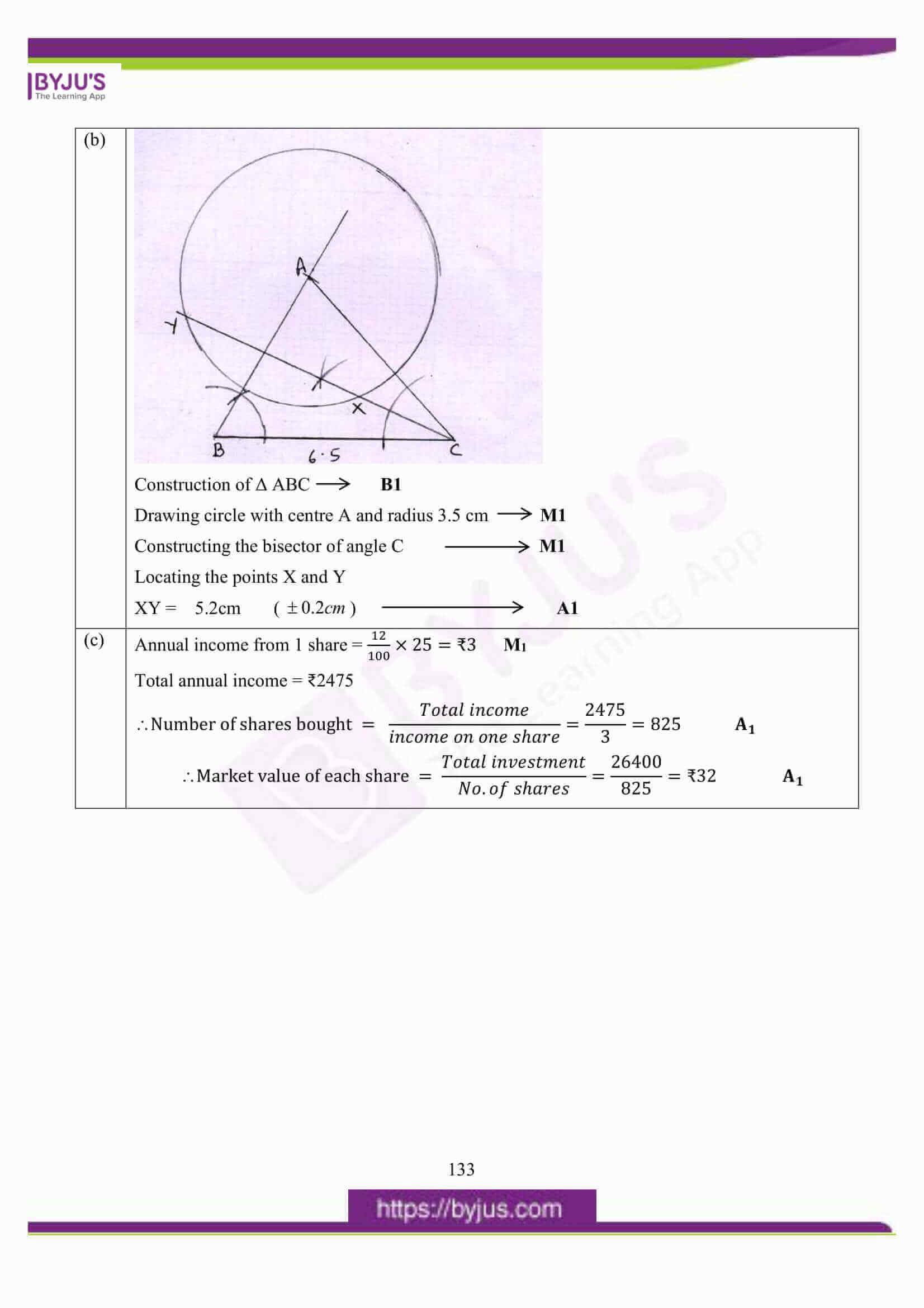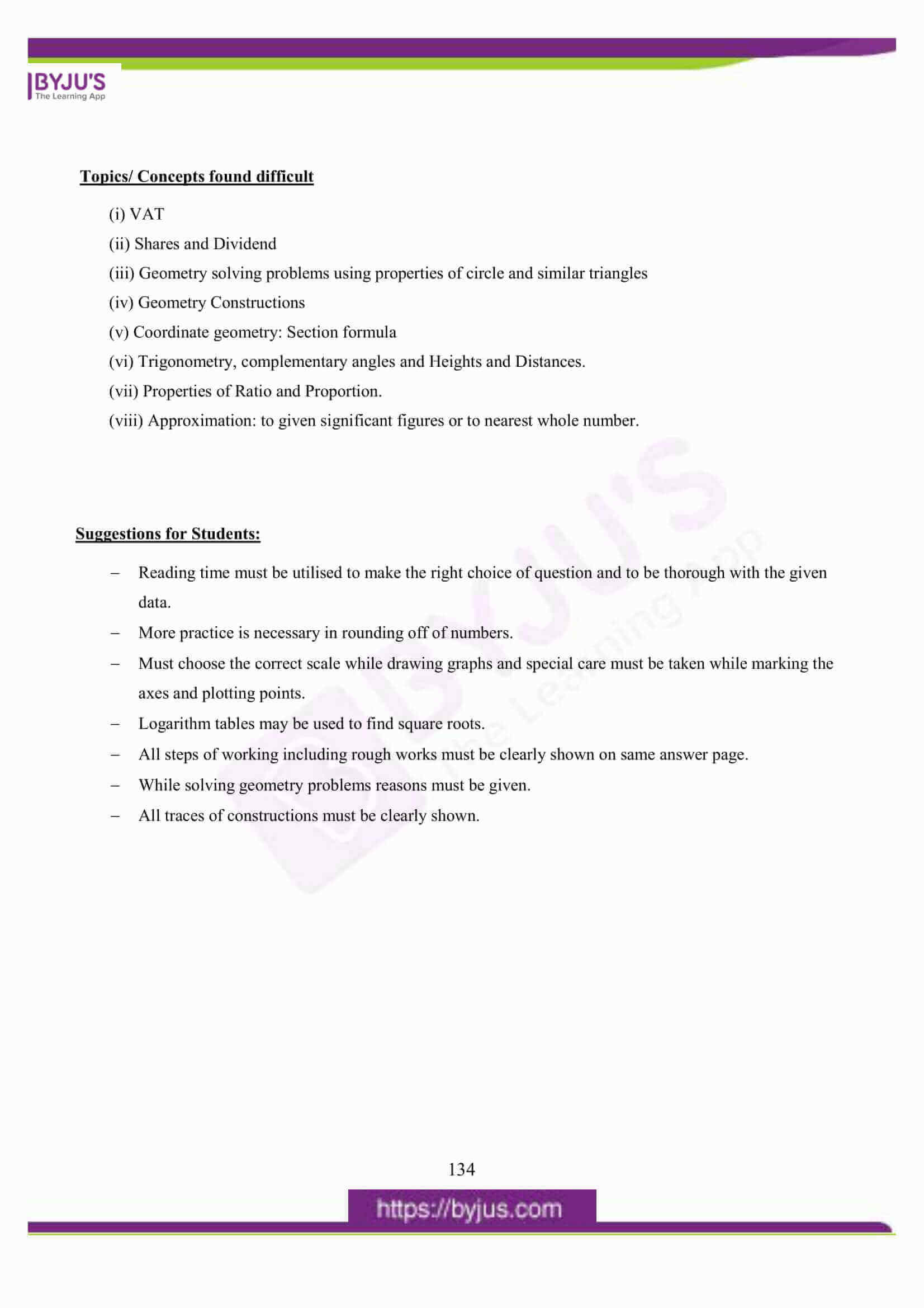## ICSE Class 10 Maths Question Paper 2016 With Solution

### Question 1:

(a) Using remainder theorem, find the value of k if on dividing 2𝑥3 + 3𝑥2 − 𝑘𝑥 + 5 by x – 2, leaves a remainder 7.

(b)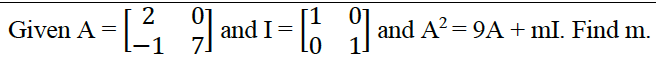(c) The mean of following numbers is 68. Find the value of ‘x’.

45, 52, 60, x, 69, 70, 26, 81 and 94.

Hence estimate the median.

(a) 𝑓(𝑥) = 2𝑥3 + 3𝑥2 − 𝑘𝑥 + 5

𝑓(2) = 2 × 23 + 3 × 22 − 𝑘 × 2 + 5 M1 (Substitution)

16 + 12 − 2𝑘 + 5 = 7 M1 (equated to 7)

33 − 2𝑘 = 7

∴ 2𝑘 = 33 − 7

2𝑘 = 26

∴ 𝑘 = 13

(b)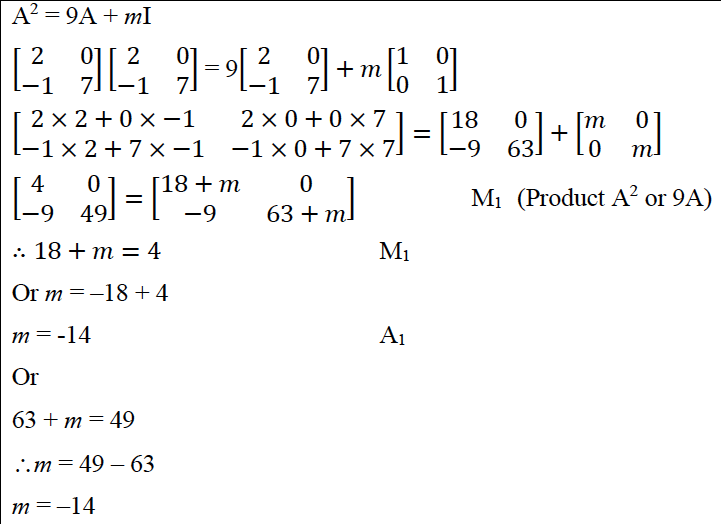(c)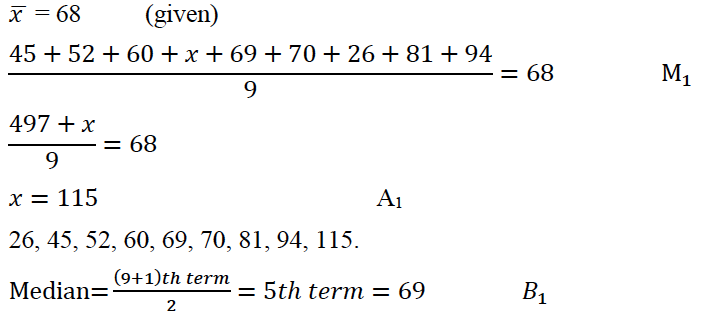### Question 2:

(a) The slope of a line joining P(6, k) and Q(1-3k, 3) is 1/2. Find

(i) k

(ii) Midpoint of PQ, using the value of ‘k’ found in (i).

(b) Without using trigonometrical tables, evaluate:

Cosec2 570 − tan2 330 + cos 440 cosec 460 − √2cos450 − tan2 600

(c) A certain number of metallic cones, each of radius 2 cm and height 3 cm are melted and recast into a solid sphere of radius 6cm. Find the number of cones.

(a)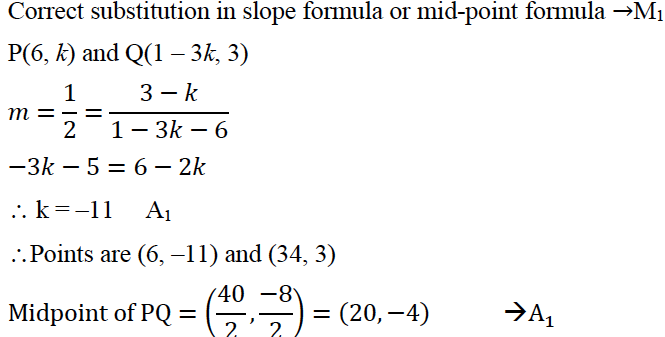(b)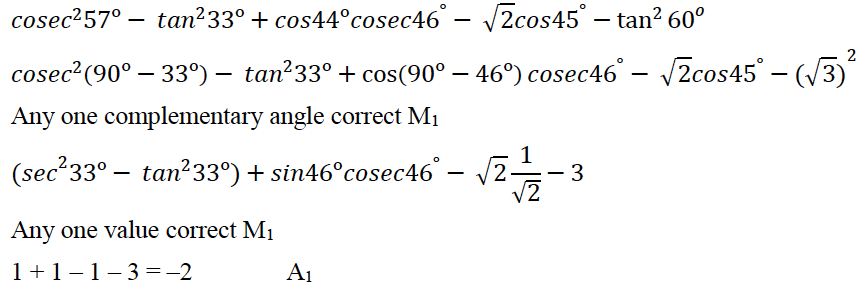(c)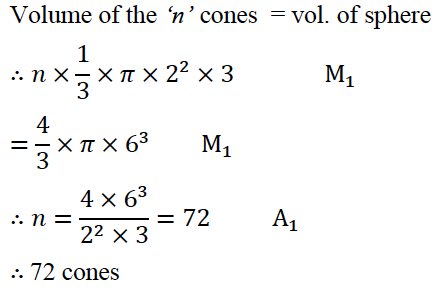### Question 3:

(a) Solve the following inequation, write the solution set and represent it on the number line.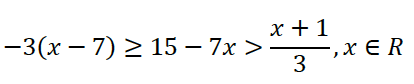(b) In the figure given below, AD is a diameter. O is the center of the circle. AD is parallel to BC and ∠CBD = 320. Find:

(i) ∠OBD

(ii) ∠AOB

(iii) ∠BED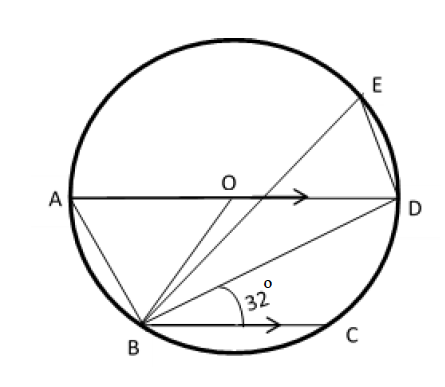(c) If (𝟑𝒂 + 𝟐𝒃): (𝟓𝒂 + 𝟑𝒃) = 𝟏𝟖: 𝟐𝟗. Find a : b.

(a)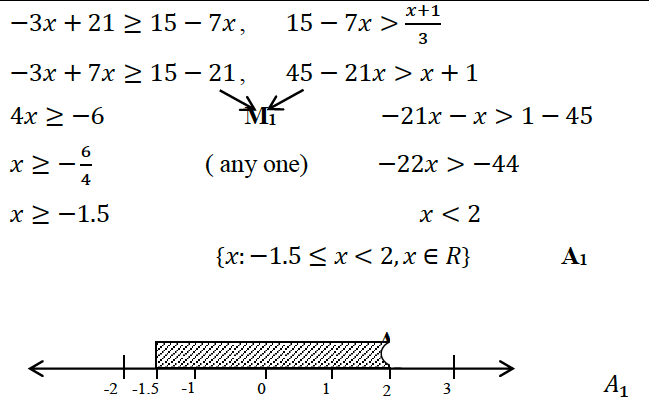(b)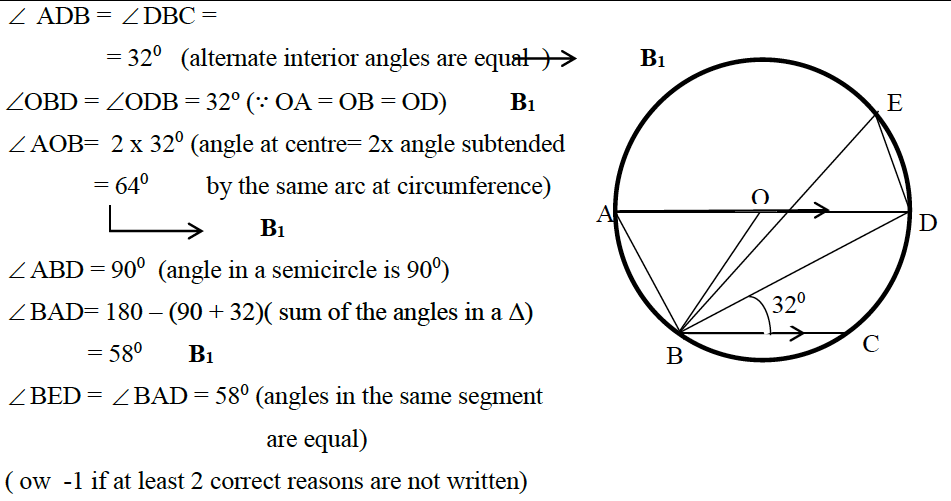(c)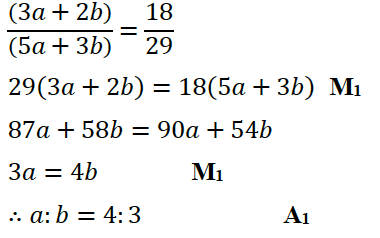### Question 4:

(a) A game of numbers has cards marked with 11, 12, 13, ….. , 40. A card is drawn at random. Find the Probability that the number on the card drawn is:

(i) A perfect square

(ii) Divisible by 7

(b) Use graph paper for this question.

(Take 2 cm = 1 unit along both x and y axis.)

Plot the points O (0, 0), A (–4, 4), B (–3, 0) and C (0, –3)

(i) Reflect points A and B on the y axis and name them A’ and B’ respectively. Write down their coordinates.

(ii) Name the figure OABCB’A’.

(iii) State the line of symmetry of this figure.

(c) Mr. Lalit invested ₹5000 at a certain rate of interest, compounded annually for two years. At the end of first year it amounts to ₹5325. Calculate

(i) The rate of interest.

(ii) The amount at the end of second year, to the nearest rupee.

(a)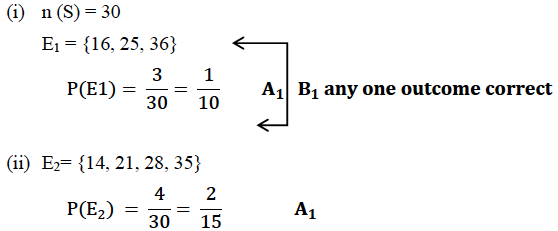(b)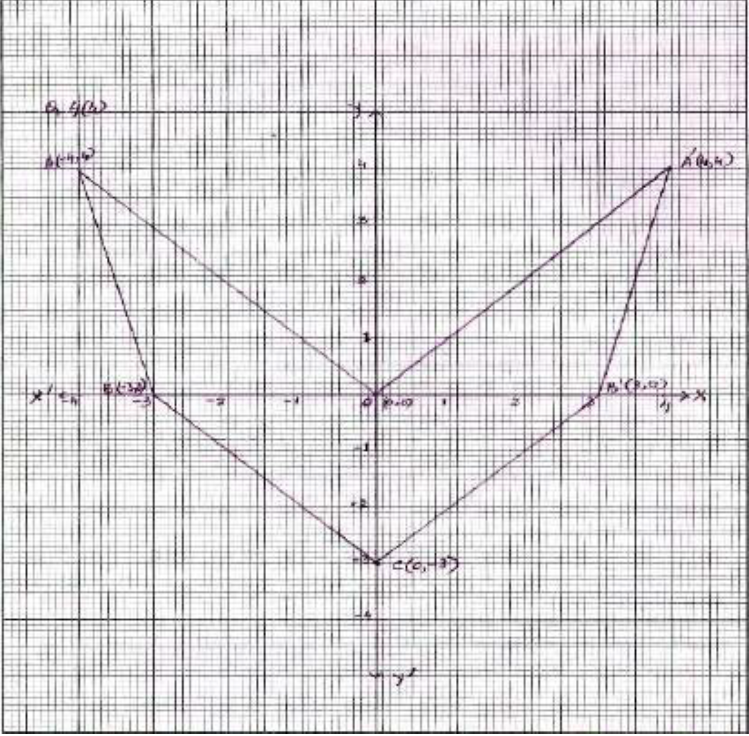(c)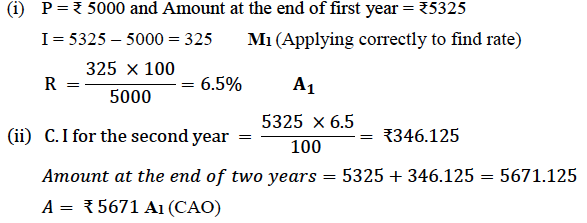### Question 5:

(a) Solve the quadratic equation 𝑥2 − 3(𝑥 + 3) = 0; Give your answer correct to two significant figures.

(b) A page from the savings bank account of Mrs. Ravi is given below.

 Date Particulars Withdrawal (₹) Deposit (₹) Balance (₹) April 3rd 2006 B/F 6000 April 7th By cash 2300 8300 April 15th By cheque 3500 11800 May 20th To self 4200 7600 June 10th By cash 5800 13400 June 15th To self 3100 10300 August 13th By cheque 1000 11300 August 25th To self 7400 3900 September 6th 2006 By cash 2000 5900

She closed the account on 30th September, 2006. Calculate the interest Mrs. Ravi earned at the end of 30th September, 2006 at 4.5% per annum interest. Hence, find the amount she receives on closing the account.

(c) In what time will Rs.1500 yield Rs.1996.50 as compound interest at 10% per annum compounded annually?

(a)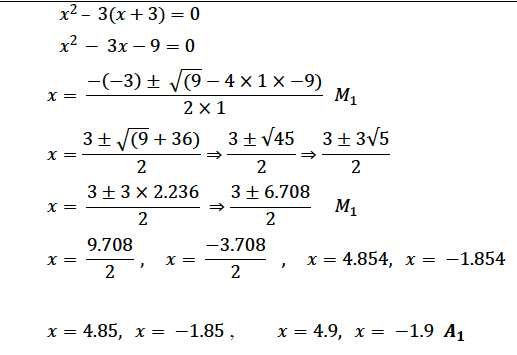(b)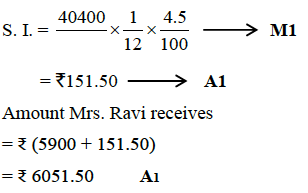(c)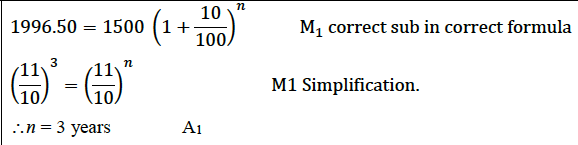### Question 6:

(a) Construct a regular hexagon of side 5 cm. Hence construct all its lines of symmetry and name them.

(b) In the given figure PQRS is a cyclic quadrilateral PQ and SR produced meet at T.

(i) Prove TPS TRQ.

(ii) Find SP if TP = 18cm, RQ = 4 cm and TR = 6cm.

(iii) Find area of quadrilateral PQRS if area of PTS = 27 cm2.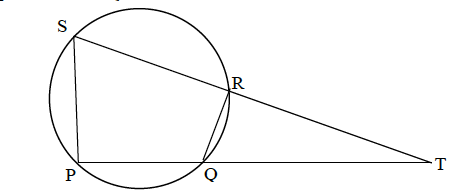(c)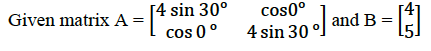If AX =B

(i) Write the order of matrix X.

(ii) Find the matrix ‘X’.

(a) Construction of one side one angle M1

Hexagon A1

Lines of symmetry any 2 (one side one angle) B1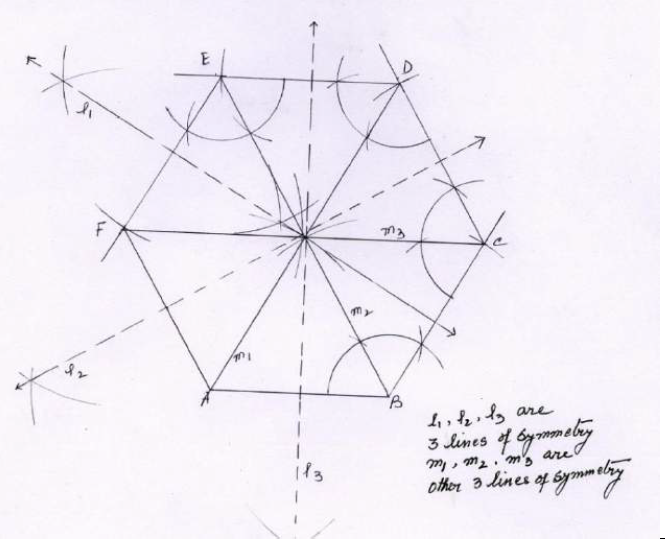(b)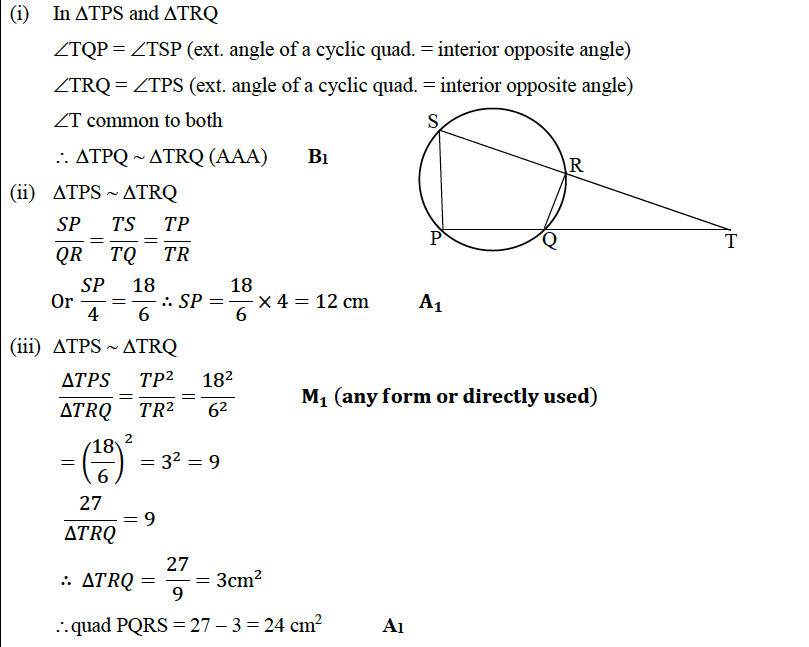(c)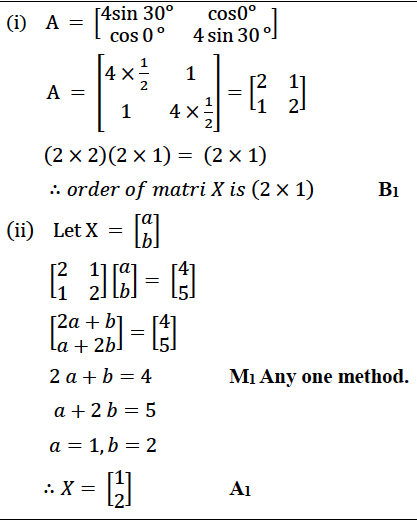### Question 7:

(a) An aeroplane at an altitude of 1500 meters finds that two ships are sailing towards it in the same direction. The angles of depression as observed from the aeroplane are 45o and 30o respectively. Find the distance between the two ships.

(b) The table shows the distribution of the scores obtained by 160 shooters in a shooting competition. Use a graph sheet and draw an ogive for the distribution. (Take 2cm = 10 scores on the X axis and 2cm = 20 shooters on the Y-axis).

 Scores 0-10 10-20 20-30 30-40 40-50 50-60 60-70 70-80 80-90 90-100 No. of Shooters 9 13 20 26 30 22 15 10 8 7

Use your graph to estimate the following:

(i) The median.

(ii) The interquartile range.

(iii) The number of shooters who obtained a score of more than 85%.

(a)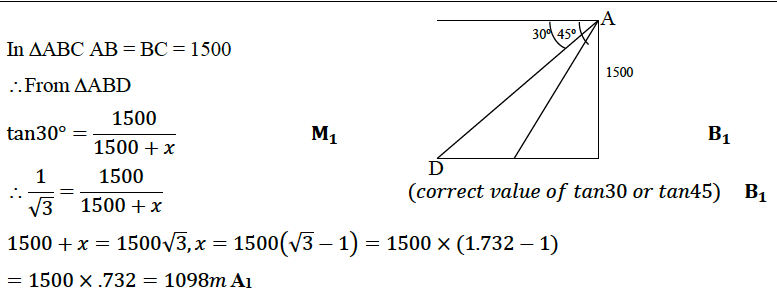(b)

c.f: 9, 22, 42, 68, 98, 120, 135, 145, 153, 160 → B1 (first 6 correct)

(For smooth S curve plotted with upper boundaries) → B1

Median = Nth/2 term. = 80th term = 44 ( ± 1) → A1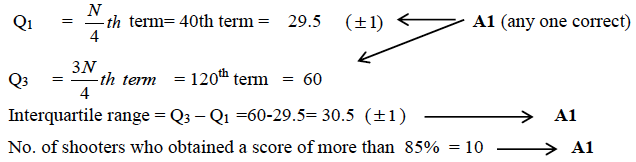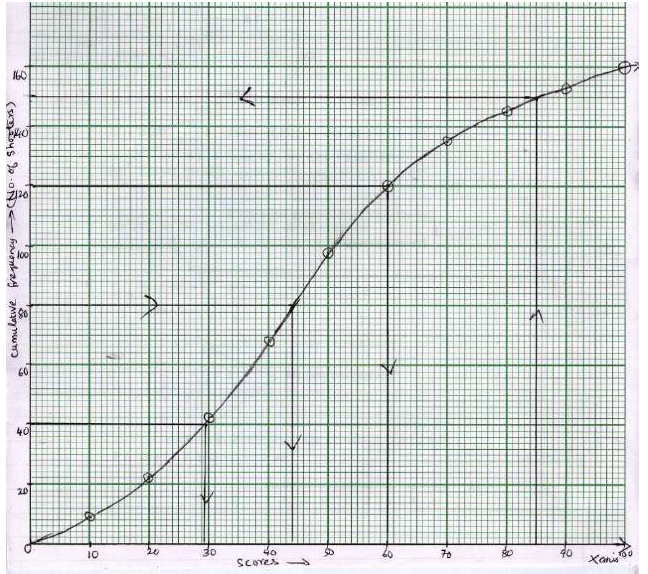### Question 8:

(a)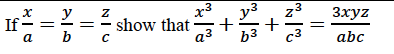(b) Draw a line AB = 5 cm. Mark a point C on AB such that AC = 3 cm. Using a ruler and a compass only, construct:

(i) A circle of radius 2.5 cm, passing through A and C.

(ii) Construct two tangents to the circle from the external point B. Measure and record the length of the tangents.

(c) A line AB meets X-axis at A and Y- axis at B. P(4, -1) divides AB in the ratio 1:2.

(i) Find the coordinates of A and B.

(ii) Find the equation of the line through P and perpendicular to AB.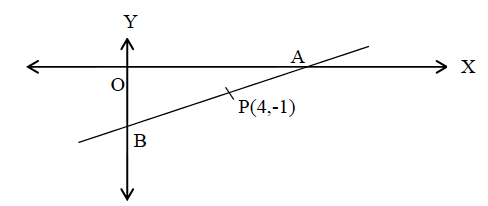(a)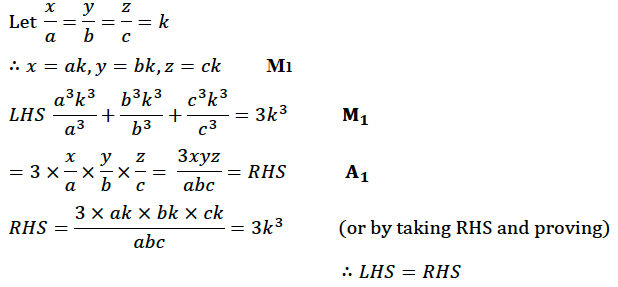(b)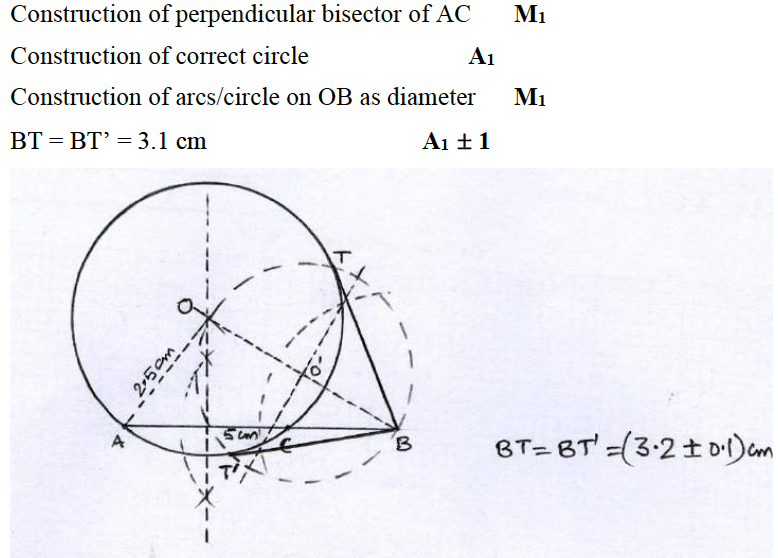(c)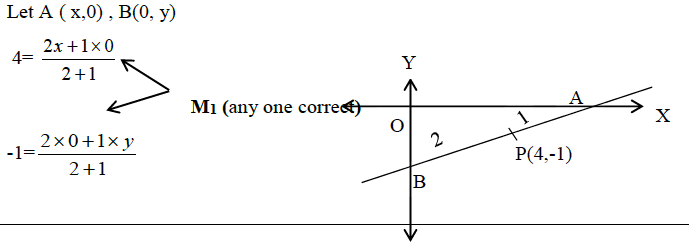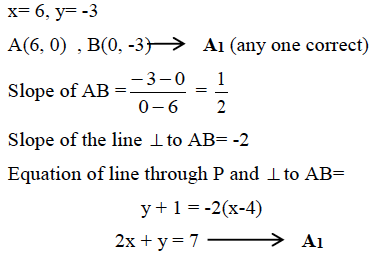### Question 9:

(a) A dealer buys an article at a discount of 30% from the wholesaler, the marked price being ₹6,000. The dealer sells it to a shopkeeper at a discount of 10% on the marked price. If the rate of VAT is 6%, find

(i) The price paid by the shopkeeper including the tax.

(ii) The VAT paid by the dealer.

(b) The given figure represents a kite with a circular and a semicircular motifs stuck on it. The radius of circle is 2.5 cm and the semicircle is 2 cm. If diagonals AC and BD are of lengths 12 cm and 8 cm respectively, find the area of the: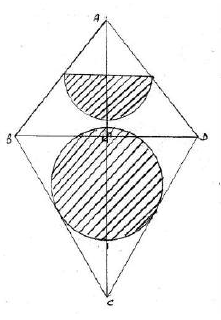(c) A model of a ship is made to a scale 1 : 300

(i) The length of the model of the ship is 2 m. Calculate the length of the ship.

(ii) The area of the deck ship is 180,000 m2. Calculate the area of the deck of the model.

(iii) The volume of the model is 6.5 m3. Calculate the volume of the ship.

(a)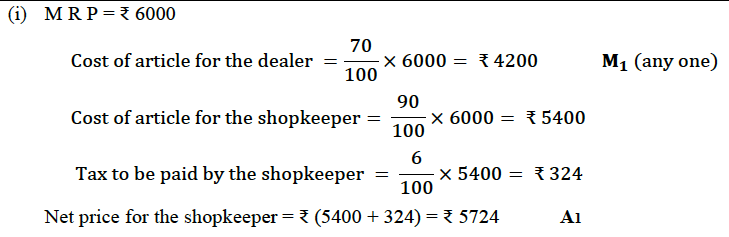(ii) Value added by the dealer = ₹ (5400 – 4200) = ₹ 1200

∴ 𝑉𝐴𝑇 𝑝𝑎𝑖𝑑 𝑏𝑦 𝑡ℎ𝑒 𝑑𝑒𝑎𝑙𝑒𝑟 = 6% 𝑜𝑓 ₹1200 = ₹ 72 A1

(b)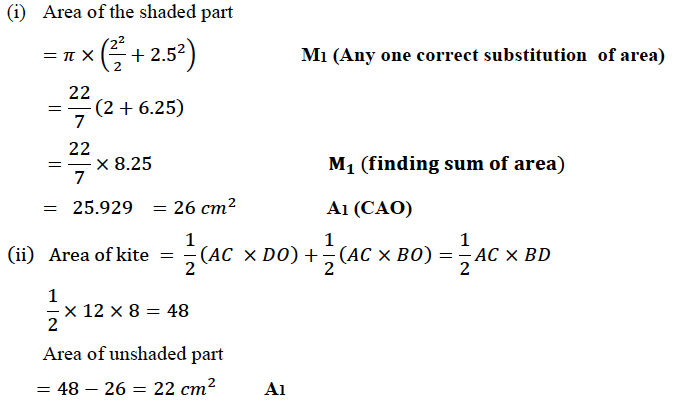(c)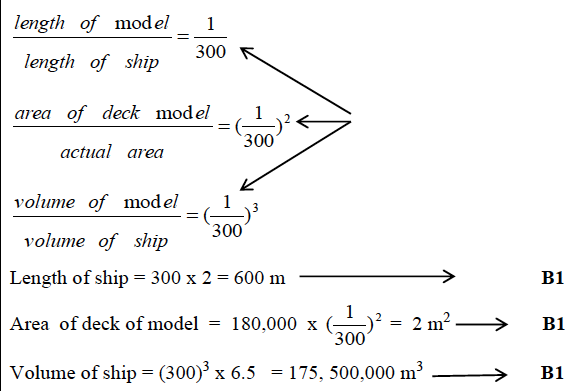### Question 10:

(a) Mohan has a recurring deposit account in a bank for 2 years at 6 % p.a. Simple interest. If he gets  1200 as interest at the time of maturity, find:

(i) the monthly instalment

(ii) the amount of maturity.

(b) The histogram below represents the scores obtained by 25 students in a Mathematics mental test. Use the data to:

(i) Frame a frequency distribution table.

(ii) To calculate mean.

(iii) To determine the Modal class.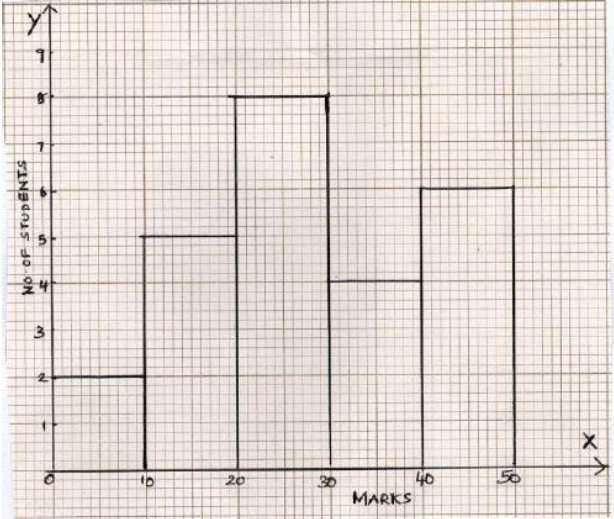(c) A bus covers a distance of 240 km at a uniform speed. Due to heavy rain its speed gets reduced by 10 km/h and as such it takes two hrs longer to cover the total distance. Assuming the uniform speed to be ‘x’ km/h, form an equation and solve it to evaluate ‘x’.

(a)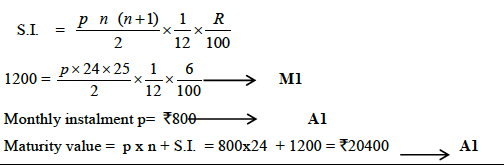(b)

 CI f x A=25D= x-A Fd 0-10 2 5 -20 -40 10-20 5 15 -10 -50 20-30 8 25 0 0 30-40 4 35 10 40 40-50 6 45 20 120 25 70

Mean = 25 + 70/25

= 25 + 2.8 = 27.8

Any 3 CI vs f correct M1

Substituting in correct formula M1 (any method)

Mean = 27.8 A1

Modal class 20 – 30 B1

(c)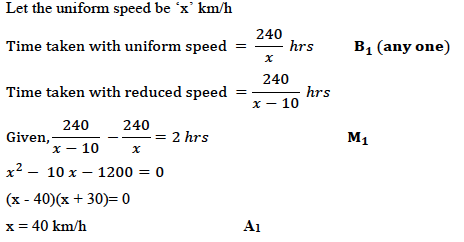### Question 11:

(a) Prove that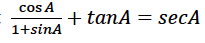(b) Use ruler and compasses only for the following question. All construction lines and arcs must be clearly shown.

(i) Construct a Δ ABC in which BC= 6.5 cm, ABC = 60o, AB= 5cm .

(ii) Construct the locus of points at a distance of 3.5 cm from A.

(iii) Construct the locus of points equidistant from AC and BC.

(iv) Mark 2 points X and Y which are at a distance of 3.5cm from A and also equidistant from AC and BC. Measure XY.

(c) Ashok invested  26,400 on 12%, 25 shares of a company. If he receives a dividend of 2,475, find the:

(i) number of shares he bought

(ii) Market value of each share

(a)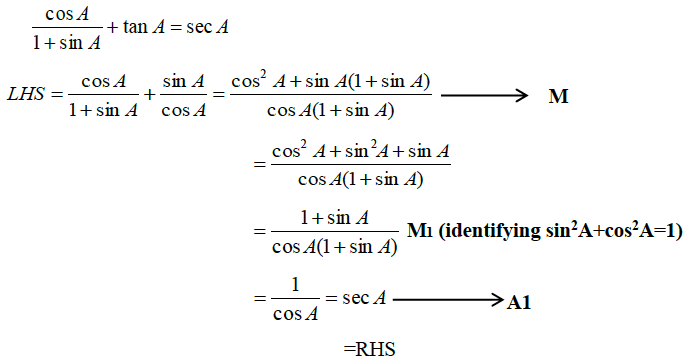(b)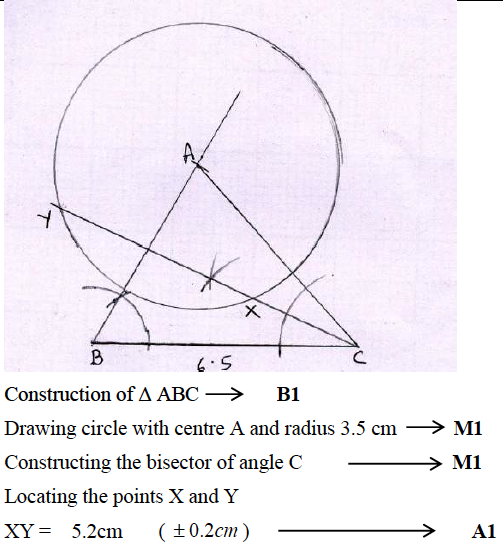(c)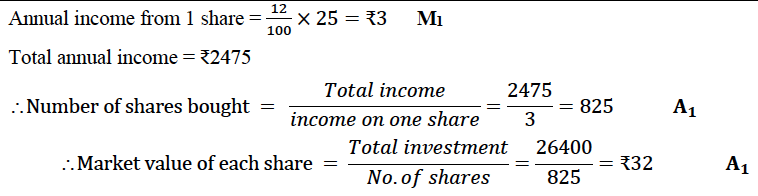We hope ICSE Class 10 Maths Question Paper Solution 2016 must have provided an insight into the 2016 Question paper and their solutions. Going through this paper solution will surely help students in their exam preparation. Students can also find the answers of other papers of ICSE Class 10 Previous Years Questions by clicking here. Happy Learning and stay tuned to BYJU’S for the latest update on ICSE/CBSE/State Boards/Competitive exams. Also, don’t forget to download the BYJU’S App.